• 面板数据(Panel Data)是将“截面数据”和“时间序列数据”综合起来的一种数据类型。具有“横截面”和“时间序列”两个维度，当这类数据按两个维度进行排列时，数据都排在一个平面上，与排在一条线上的一维数据有着...
        面板数据(Panel Data)是将“截面数据”和“时间序列数据”综合起来的一种数据类型。具有“横截面”和“时间序列”两个维度，当这类数据按两个维度进行排列时，数据都排在一个平面上，与排在一条线上的一维数据有着明显的不同，整个表格像是一个面板，所以称为面板数据(Panel Data)。
实际上如果从数据结构内在含义上，应该把Panel Data称为“时间序列-截面数据”，更能体现数据结构本质上的特点。该数据为也被称为“纵向数据(Longitudinal Data)”，“平行数据”，“TS-CS数据(Time Series-Cross Section)”。它是截面上个体在不同时间点的重复测量数据。面板数据从横截面(cross section)看，是由若干个体(entity,unit,individual)在某一时点构成的截面观测值，从纵剖面(longitudinal section)看每个个体都是一个时间序列。
从时空维度来看，可将计量经济学中应用的数据分三类：
1、横截面数据(Cross-sectional data)
横截面数据是指在某一时点收集的不同对象的数据。它对应同一时点上不同空间(对象)所组成的一维数据集合，研究的是某一时点上的某种经济现象，突出空间(对象)的差异。横截面数据的突出特点就是离散性高。横截面数据体现的是个体的个性，突出个体的差异，通常横截面数据表现的是无规律的而非真正的随机变化。即计量经济学中所谓的“无法观测的异质性”。在分析横截面数据时，应主要注意两个问题：一是异方差问题，由于数据是在某一时期对个体或地域的样本的采集，不同个体或地域本身就存在差异；二是数据的一致性，主要包括变量的样本容量是否一致、样本的取样时期是否一致、数据的统计标准是否一致。图片来源于网络
2、时间序列数据(Time-series data)
时间序列数据是指对同一对象在不同时间连续观察所取得的数据。它着眼于研究对象在时间顺序上的变化，寻找空间(对象)历时发展的规律。利用时间序列作样本时，要注意几个问题：一是所选择的样本区间内经济行为的一致性问题；二是样本数据在不同样本点之间不可比，需要对原始数据进行调整，消除其不可比因素；三是样本观测值过于集中，因而时间序列数据不适宜于对模型中反映长期变化关系的结构参数的估计；四是模型随机误差的序列相关问题。图片来源于网络
3、纵向数据(Longitudinal data)或面板数据(Panel data)
面板数据，即Panel Data，是截面数据与时间序列综合起来的一种数据资源。 在分析时,多用PanelData模型,故也被称为面板数据模型. 它可以用于分析各样本在时间序列上组成的数据的特征,它能够综合利用样本信息,通过模型中的参数,既可以分析个体之间的差异情况,又可以描述个体的动态变化特征。图片来源于网络

举栗一个，栗子来源于网络！
时间序列数据：北京一年来每天的平均温度。
截面数据：北京，上海，深圳，广州某一天的平均温度。
面板数据：北京，上海，深圳，广州这一年来每天的平均温度。
适用范围：
时间序列数据：某一个个体随时间变化产生的数据。
截面数据：许多个个体在同一个时间下由于个体不同而产生的数据。
面板数据：前两个的综合——许多个个体由于个体不同以及时间变化产生的数据。
分析方法：
时间序列数据：主要围绕时间变化，可看总体趋势，季节性，周期性，ARIMA（自回归，滑动平均，差分）等。
截面数据：主要围绕统计个体区别，可用线性回归，主元分析等。 面板数据：前两个的综合。

举栗二个，栗子来源于网络！
如：城市名：北京、上海、重庆、天津的GDP分别为10、11、9、8（单位亿元）。这就是截面数据，在一个时间点处切开，看各个城市的不同就是截面数据。
如：2000、2001、2002、2003、2004各年的北京市GDP分别为8、9、10、11、12（单位亿元）。这就是时间序列，选一个城市，看各个样本时间点的不同就是时间序列。
如：2000、2001、2002、2003、2004各年中国所有直辖市的GDP分别为：
北京市分别为8、9、10、11、12；
上海市分别为9、10、11、12、13；
天津市分别为5、6、7、8、9；
重庆市分别为7、8、9、10、11（单位亿元）。
这就是面板数据。
展开全文横截面数据 面板数据 纵向数据
• 时间序列是按照一定的时间间隔排列的一组数据，其时间间隔可以是任意的时间单位，如小时、日、周月等。比如，每天某产品的用户数量，每个月的销售额，这些数据形成了一定时间间隔的数据。 通过对这些时间序列的...
时间序列系列文章：
时间序列（一）：时间序列数据与时间序列预测模型 时间序列（二）：时间序列平稳性检测 时间序列（三）：ARIMA模型实战
时间序列及其预测是日常工作中建模，分析，预测的重要组成部分。本系列我们将从0开始介绍时间序列的含义，模型及其分析。本篇为第一部分，我们主要介绍时间序列，与其常用的预测模型。
时间序列定义：
时间序列是按照一定的时间间隔排列的一组数据，其时间间隔可以是任意的时间单位，如小时、日、周月等。比如，每天某产品的用户数量，每个月的销售额，这些数据形成了以一定时间间隔的数据。
通过对这些时间序列的分析，从中发现和揭示现象发展变化的规律，并将这些知识和信息用于预测。比如销售量是上升还是下降，销售量是否与季节有关，是否可以通过现有的数据预测未来一年的销售额是多少等。
对于时间序列的预测，由于很难确定它与其他变量之间的关系，这时我们就不能用回归去预测，而应使用时间序列方法进行预测。
采用时间序列分析进行预测时需要一系列的模型，这种模型称为时间序列模型。
时间序列预测模型与方法
注：本部分只关注相关模型与分析的方法，模型的选择，调参与优化会放在后续文章中详细讲解
原始数据
本文所使用原始数据与代码，可以在公众号：Smilecoc的杂货铺 中回复“时间序列”获取。可直接扫描文末二维码关注！
朴素法
朴素法就是预测值等于实际观察到的最后一个值。它假设数据是平稳且没有趋势性与季节性的。通俗来说就是以后的预测值都等于最后的值。
这种方法很明显适用情况极少，所以我们重点通过这个方法来熟悉一下数据可视化与模型的评价及其相关代码。
#朴素法
dd = np.asarray(train['Count'])#训练组数据
y_hat = test.copy()#测试组数据
y_hat['naive'] = dd[len(dd) - 1]#预测组数据

#数据可视化
plt.figure(figsize=(12, 8))
plt.plot(train.index, train['Count'], label='Train')
plt.plot(test.index, test['Count'], label='Test')
plt.plot(y_hat.index, y_hat['naive'], label='Naive Forecast')
plt.legend(loc='best')
plt.title("Naive Forecast")
plt.show()

得到结果：我们通过计算均方根误差，检查模型在测试数据集上的准确率。 其中均方根误差（RMSE）是各数据偏离真实值的距离平方和的平均数的开方
#计算均方根误差RMSE
from sklearn.metrics import mean_squared_error
from math import sqrt

# mean_squared_error求均方误差
rmse = sqrt(mean_squared_error(test['Count'], y_hat['naive']))
print(rmse)

得到均方根误差为1053
简单平均法
简单平均法就是预测的值为之前过去所有值的平均.当然这不会很准确，但这种预测方法在某些情况下效果是最好的。
#简单平均法
y_hat_avg = test.copy()
y_hat_avg['avg_forecast'] = train['Count'].mean()

其后续可视化与模型效果评估方法与上述一致，这里不再赘述，需要详细代码可以查看相关源码。得到RMSE值为2637
移动平均法
我们经常会遇到这种数据集，比如价格或销售额某段时间大幅上升或下降。如果我们这时用之前的简单平均法，就得使用所有先前数据的平均值，但在这里使用之前的所有数据是说不通的，因为用开始阶段的价格值会大幅影响接下来日期的预测值。因此，我们只取最近几个时期的价格平均值。很明显这里的逻辑是只有最近的值最要紧。这种用某些窗口期计算平均值的预测方法就叫移动平均法。
#移动平均法
y_hat_avg = test.copy()
#利用时间窗函数rolling求平均值u
y_hat_avg['moving_avg_forecast'] = train['Count'].rolling(60).mean().iloc[-1]

其后续可视化与模型效果评估方法与上述一致，这里不再赘述，需要详细代码可以查看相关源码。得到RMSE值为1121
指数平滑法
在做时序预测时，一个显然的思路是：认为离着预测点越近的点，作用越大。比如我这个月体重100斤，去年某个月120斤，显然对于预测下个月体重而言，这个月的数据影响力更大些。假设随着时间变化权重以指数方式下降——最近为0.8，然后0.8**2，0.8**3…，最终年代久远的数据权重将接近于0。将权重按照指数级进行衰减，这就是指数平滑法的基本思想。
指数平滑法有几种不同形式：一次指数平滑法针对没有趋势和季节性的序列，二次指数平滑法针对有趋势但没有季节性的序列，三次指数平滑法针对有趋势也有季节性的序列。“
所有的指数平滑法都要更新上一时间步长的计算结果，并使用当前时间步长的数据中包含的新信息。它们通过”混合“新信息和旧信息来实现，而相关的新旧信息的权重由一个可调整的参数来控制。
一次指数平滑
一次指数平滑法的递推关系如下：

s

i

=

α

x

i

+

(

1

−

α

)

s

i

−

1

，

其

中

0

≤

α

≤

1

s_{i}=\alpha x_{i}+(1-\alpha)s_{i-1}，其中 0 \leq \alpha \leq 1

其中，

s

i

s_{i}

是时间步长i（理解为第i个时间点）上经过平滑后的值，

x

i

x_{i}

是这个时间步长上的实际数据。

α

\alpha

可以是0和1之间的任意值，它控制着新旧信息之间的平衡：当

α

\alpha

接近1，就只保留当前数据点；当

α

\alpha

接近0时，就只保留前面的平滑值(整个曲线都是平的)。我们展开它的递推关系式：
我们展开它的递推关系式：

s

i

=

α

x

i

+

(

1

−

α

)

s

i

−

1

=

α

x

i

+

(

1

−

α

)

[

α

x

i

−

1

+

(

1

−

α

)

s

i

−

2

]

=

α

x

i

+

(

1

−

α

)

[

α

x

i

−

1

+

(

1

−

α

)

[

α

x

i

−

2

+

(

1

−

α

)

s

i

−

3

]

]

=

α

[

x

i

+

(

1

−

α

)

x

i

−

1

+

(

1

−

α

)

2

x

i

−

2

+

(

1

−

α

)

3

s

i

−

3

]

=

.

.

.

=

α

∑

j

=

0

i

(

1

−

α

)

j

x

i

−

j

\begin{aligned} s_{i}&=\alpha x_{i}+(1-\alpha)s_{i-1} \\ &=\alpha x_{i}+(1-\alpha)[\alpha x_{i-1}+(1-\alpha)s_{i-2}]\\ &=\alpha x_{i}+(1-\alpha)[\alpha x_{i-1}+(1-\alpha)[\alpha x_{i-2}+(1-\alpha)s_{i-3}]]\\ &=\alpha[x_{i}+(1-\alpha)x_{i-1}+(1-\alpha)^{2}x_{i-2}+(1-\alpha)^{3}s_{i-3}]\\ &=... \\ &=\alpha\sum_{j=0}^{i}(1-\alpha)^{j}x_{i-j} \end{aligned}

可以看出，在指数平滑法中，所有先前的观测值都对当前的平滑值产生了影响，但它们所起的作用随着参数

α

\alpha

的幂的增大而逐渐减小。那些相对较早的观测值所起的作用相对较小。同时，称α为记忆衰减因子可能更合适——因为α的值越大，模型对历史数据“遗忘”的就越快。从某种程度来说，指数平滑法就像是拥有无限记忆（平滑窗口足够大）且权值呈指数级递减的移动平均法。一次指数平滑所得的计算结果可以在数据集及范围之外进行扩展，因此也就可以用来进行预测。预测方式为：

x

i

+

h

=

s

i

x_{i+h}=s_{i}

s

i

s_{i}

是最后一个已经算出来的值。h等于1代表预测的下一个值。
我们可以通过statsmodels中的时间序列模型进行指数平滑建模。官方文档地址为： https://www.statsmodels.org/stable/generated/statsmodels.tsa.holtwinters.SimpleExpSmoothing.html 具体代码如下：
#一次指数平滑
from statsmodels.tsa.api import SimpleExpSmoothing

y_hat_avg = test.copy()
fit = SimpleExpSmoothing(np.asarray(train['Count'])).fit(smoothing_level=0.6, optimized=False)
y_hat_avg['SES'] = fit.forecast(len(test))

之后同样进行数据可视化并查看模型效果
plt.figure(figsize=(16, 8))
plt.plot(train['Count'], label='Train')
plt.plot(test['Count'], label='Test')
plt.plot(y_hat_avg['SES'], label='SES')
plt.legend(loc='best')
plt.show()

可视化结果为：RMSE结果为1040
二次指数平滑
在介绍二次指数平滑前介绍一下趋势的概念。
趋势，或者说斜率的定义很简单：

b

=

Δ

y

/

Δ

x

b=Δy/Δx

，其中

Δ

x

Δx

为两点在x坐标轴的变化值，所以对于一个序列而言，相邻两个点的

Δ

x

=

1

Δx=1

，因此

b

=

Δ

y

=

y

(

x

)

−

y

(

x

−

1

)

b=Δy=y(x)-y(x-1)

s

i

=

α

x

i

+

(

1

−

α

)

(

s

i

−

1

+

t

i

−

1

)

s_{i}=\alpha x_{i}+(1-\alpha)(s_{i-1}+t_{i-1})

趋势等式

t

i

=

β

(

s

i

−

s

i

−

1

)

+

(

1

−

β

)

t

i

−

1

t_{i}=\beta (s_{i}-s_{i-1})+(1-\beta)t_{i-1}

第二个等式描述了平滑后的趋势。当前趋势的未平滑“值”（

t

i

t_{i}

）是当前平滑值（

s

i

s_{i}

）和上一个平滑值（

s

i

−

1

s_{i-1}

）的差；也就是说，当前趋势告诉我们在上一个时间步长里平滑信号改变了多少。要想使趋势平滑，我们用一次指数平滑法对趋势进行处理，并使用参数

β

\beta

（理解：对

t

i

t_{i}

的处理类似于一次平滑指数法中的

s

i

s_{i}

，即对趋势也需要做一个平滑，临近的趋势权重大）。
为获得平滑信号，我们像上次那样进行一次混合，但要同时考虑到上一个平滑信号及趋势。假设单个步长时间内保持着上一个趋势，那么第一个等式的最后那项就可以对当前平滑信号进行估计。
若要利用该计算结果进行预测，就取最后那个平滑值，然后每增加一个时间步长就在该平滑值上增加一次最后那个平滑趋势：

x

i

+

h

=

s

i

+

h

t

i

x_{i+h}=s_{i}+ht_{i}之后使用二次指数平滑进行预测：
from statsmodels.tsa.api import Holt

y_hat_avg = test.copy()

fit = Holt(np.asarray(train['Count'])).fit(smoothing_level=0.3, smoothing_slope=0.1)
y_hat_avg['Holt_linear'] = fit.forecast(len(test))

结果如图：得到对应的RMSE为1033
三次指数平滑
在应用这种算法前，我们先介绍一个新术语。假如有家酒店坐落在半山腰上，夏季的时候生意很好，顾客很多，但每年其余时间顾客很少。因此，每年夏季的收入会远高于其它季节，而且每年都是这样，那么这种重复现象叫做“季节性”（Seasonality）。如果数据集在一定时间段内的固定区间内呈现相似的模式，那么该数据集就具有季节性。二次指数平滑考虑了序列的基数和趋势，三次就是在此基础上增加了一个季节分量。类似于趋势分量，对季节分量也要做指数平滑。比如预测下一个季节第3个点的季节分量时，需要指数平滑地考虑当前季节第3个点的季节分量、上个季节第3个点的季节分量…等等。详细的有下述公式(累加法)：

s

i

=

α

(

x

i

−

p

i

−

k

)

+

(

1

−

α

)

(

s

i

−

1

+

t

i

−

1

)

t

i

=

β

(

s

i

−

s

i

−

1

)

+

(

1

−

β

)

t

i

−

1

p

i

=

γ

(

x

i

−

s

i

)

+

(

1

−

γ

)

p

i

−

k

\begin{aligned} s_{i}&=\alpha (x_{i}-p_{i-k})+(1-\alpha)(s_{i-1}+t_{i-1}) \\ t_{i} &=\beta (s_{i}-s_{i-1})+(1-\beta)t_{i-1}\\ p_{i}&=\gamma (x_{i}-s_{i})+(1-\gamma)p_{i-k} \end{aligned}

其中，

p

i

p_{i}

是指“周期性”部分。预测公式如下：

x

i

+

h

=

s

i

+

h

t

i

+

p

i

−

k

+

h

x_{i+h}=s_{i}+ht_{i}+p_{i-k+h}

k 是这个周期的长度。
在使用二次平滑模型与三次平滑模型前，我们可以使用sm.tsa.seasonal_decompose分解时间序列，可以得到以下分解图形——从上到下依次是原始数据、趋势数据、周期性数据、随机变量（残差值）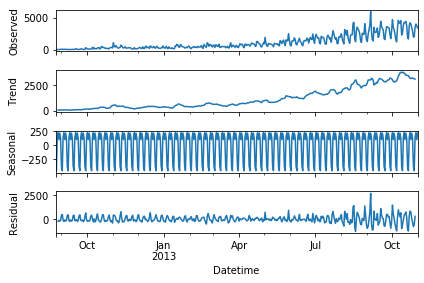根据分析图形和数据可以确定对应的季节参数
具体代码为：
#三次指数平滑
from statsmodels.tsa.api import ExponentialSmoothing

y_hat_avg = test.copy()
y_hat_avg['Holt_Winter'] = fit1.forecast(len(test))得到的RMSE为575。我们可以看到趋势和季节性的预测准确度都很高。你可以试着调整参数来优化这个模型。
AR模型
AR(Auto Regressive Model)自回归模型是线性时间序列分析模型中最简单的模型。通过自身前面部分的数据与后面部分的数据之间的相关关系（自相关）来建立回归方程，从而可以进行预测或者分析。服从p阶的自回归方程表达式如下：

x

t

=

ϕ

1

x

t

−

1

+

ϕ

2

x

t

−

2

+

⋯

+

ϕ

p

x

t

−

p

+

μ

t

x_{t}=\phi_{1}x_{t-1}+\phi_{2}x_{t-2}+\cdots+\phi_{p}x_{t-p}+\mu_{t}

表示为

A

R

(

p

)

AR(p)

,。其中，

μ

t

\mu_{t}

表示白噪声，是时间序列中的数值的随机波动，但是这些波动会相互抵消，最终是0。

ϕ

\phi

表示自回归系数。
所以当只有一个时间记录点时，称为一阶自回归过程，即AR(1)。其表达式为：

x

t

=

ϕ

1

x

t

−

1

+

μ

t

x_{t}=\phi_{1}x_{t-1}+\mu_{t}

利用Python建立AR模型一般会用到我们之后会说到的ARIMA模型（AR模型中的p是ARIMA模型中的参数之一，只要将其他的参数设置为0即为AR模型）。您可以先阅读后续ARIMA模型的内容并参考文件中的代码查看具体的内容
MA模型
MA(Moving Average Model)移动平均模型通过将一段时间序列中白噪声(误差)进行加权和，可以得到移动平均方程。如下模型为q阶移动平均过程，表示为MA(q)。

x

t

=

μ

+

μ

t

+

θ

1

μ

t

−

1

+

θ

2

μ

t

−

2

+

⋯

+

θ

q

μ

t

−

q

x_{t}=\mu+\mu_{t}+\theta_{1}\mu_{t-1}+\theta_{2}\mu_{t-2}+\cdots+\theta_{q}\mu_{t-q}

其中

x

t

x_{t}

表示t期的值，当期的值由前q期的误差值来决定，

μ

μ

值是常数项，相当于普通回归中的截距项，

μ

t

\mu_{t}

是当期的随机误差。MA模型的核心思想是每一期的随机误差都会影响当期值，把前q期的所有误差加起来就是对t期值的影响。
同样，利用Python建立MA模型一般会用到我们之后会说到的ARIMA模型,您可以先阅读后续ARIMA模型的内容并参考文件中的代码查看具体的内容
ARMA模型
ARMA(Auto Regressive and Moving Average Model)自回归移动平均模型是与自回归和移动平均模型两部分组成。所以可以表示为ARMA(p, q)。p是自回归阶数，q是移动平均阶数。

x

t

=

ϕ

1

x

t

−

1

+

ϕ

2

x

t

−

2

+

⋯

+

ϕ

p

x

t

−

p

+

μ

t

+

θ

1

μ

t

−

1

+

θ

2

μ

t

−

2

+

⋯

+

θ

q

μ

t

−

q

x_{t}=\phi_{1}x_{t-1}+\phi_{2}x_{t-2}+\cdots+\phi_{p}x_{t-p}+\mu_{t}+\theta_{1}\mu_{t-1}+\theta_{2}\mu_{t-2}+\cdots+\theta_{q}\mu_{t-q}

从式子中就可以看出，自回归模型结合了两个模型的特点，其中，AR可以解决当前数据与后期数据之间的关系，MA则可以解决随机变动也就是噪声的问题。
ARIMA模型
ARIMA(Auto Regressive Integrate Moving Average Model)差分自回归移动平均模型是在ARMA模型的基础上进行改造的，ARMA模型是针对t期值进行建模的，而ARIMA是针对t期与t-d期之间差值进行建模，我们把这种不同期之间做差称为差分，这里的d是几就是几阶差分。ARIMA模型也是基于平稳的时间序列的或者差分化后是稳定的，另外前面的几种模型都可以看作ARIMA的某种特殊形式。表示为ARIMA(p, d, q)。p为自回归阶数，q为移动平均阶数，d为时间成为平稳时所做的差分次数，也就是Integrate单词的在这里的意思。
具体步骤如下：

x

t

=

ϕ

1

w

t

−

1

+

ϕ

2

w

t

−

2

+

⋯

+

ϕ

p

w

t

−

p

+

μ

t

+

θ

1

μ

t

−

1

+

θ

2

μ

t

−

2

+

⋯

+

θ

q

μ

t

−

q

x_{t}=\phi_{1}w_{t-1}+\phi_{2}w_{t-2}+\cdots+\phi_{p}w_{t-p}+\mu_{t}+\theta_{1}\mu_{t-1}+\theta_{2}\mu_{t-2}+\cdots+\theta_{q}\mu_{t-q}

上面公式中的

w

t

w_{t}

表示t期经过d阶差分以后的结果。我们可以看到ARIMA模型的形式基本与ARMA的形式是一致的，只不过把

X

X

换成了

w

w

使用ARIMA进行预测代码如下：
from statsmodels.tsa.arima_model import ARIMA

ts_ARIMA= train['Count'].astype(float)
fit1 = ARIMA(ts_ARIMA, order=(7, 1, 4)).fit()
y_hat_ARIMA = fit1.predict(start="2013-11-1", end="2013-12-31", dynamic=True)

并画出预测值与实际值图形：
plt.figure(figsize=(16, 8))
plt.plot(train['Count'], label='Train')
plt.plot(test['Count'], label='Test')
plt.plot(y_hat_ARIMA, label='ARIMA')
plt.legend(loc='best')
plt.show()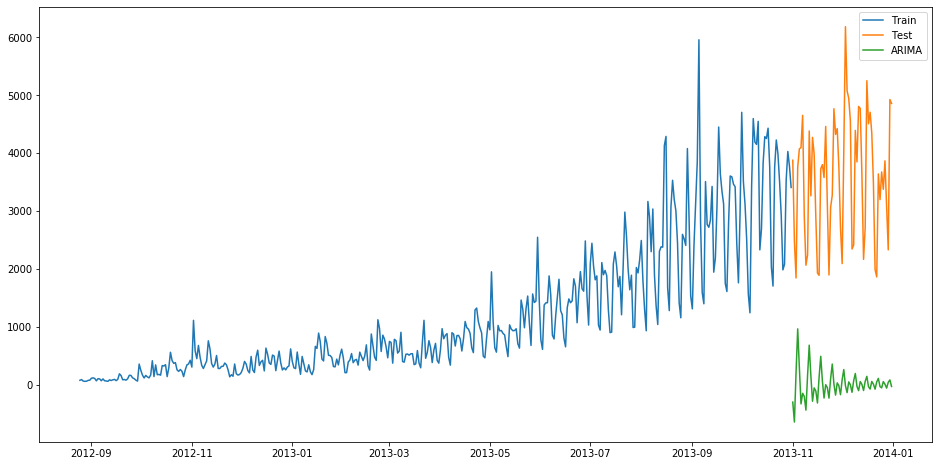并计算RMSE:
from sklearn.metrics import mean_squared_error
from math import sqrt

rmse = sqrt(mean_squared_error(test['Count'],y_hat_ARIMA.to_frame()))
print(rmse)

得到对应的RMSE为3723
SARIMA模型
SARIMA季节性自回归移动平均模型模型在ARIMA模型的基础上添加了季节性的影响，结构参数有七个：SARIMA(p,d,q)(P,D,Q,s) 其中p,d,q分别为之前ARIMA模型中我们所说的p:趋势的自回归阶数。d:趋势差分阶数。q:趋势的移动平均阶数。 P:季节性自回归阶数。 D:季节性差分阶数。 Q:季节性移动平均阶数。 s:单个季节性周期的时间步长数。
import statsmodels.api as sm
y_hat_avg = test.copy()
fit1 = sm.tsa.statespace.SARIMAX(train.Count, order=(2, 1, 4), seasonal_order=(0, 1, 1, 7)).fit()
y_hat_avg['SARIMA'] = fit1.predict(start="2013-11-1", end="2013-12-31", dynamic=True)

得到实际值与预测值如下：
plt.figure(figsize=(16, 8))
plt.plot(train['Count'], label='Train')
plt.plot(test['Count'], label='Test')
plt.plot(y_hat_avg['SARIMA'], label='SARIMA')
plt.legend(loc='best')
plt.show()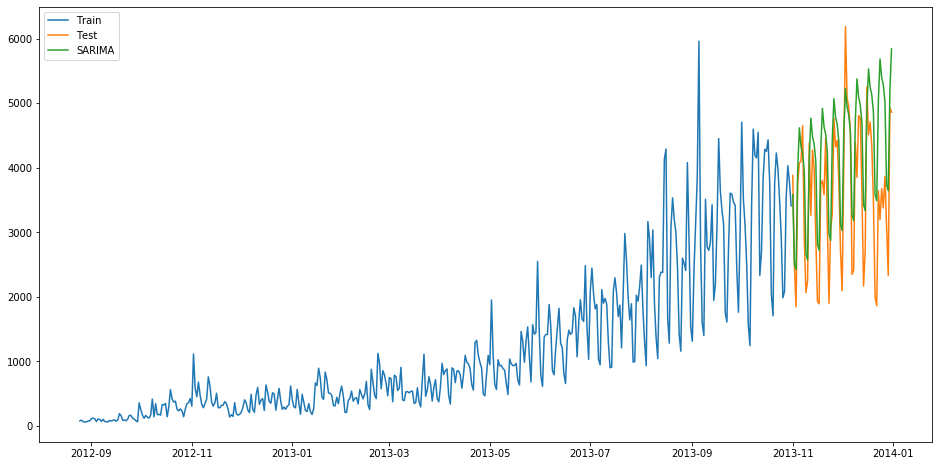并计算RMSE：
from sklearn.metrics import mean_squared_error
from math import sqrt
rmse = sqrt(mean_squared_error(test['Count'], y_hat_avg['SARIMA']))
print(rmse)

结果为933
其他时间序列预测的模型还有SARIMAX模型（在ARIMA模型上加了季节性的因素），Prophet模型，ARCH模型，LSTM神经网络模型等。限于篇幅，感兴趣的同学可以自行查看相关模型资料
在后续的文章中我们将讲解如何确定数据的平稳性与数据预处理，为后续时间序列的建模做准备
参考文章： https://www.analyticsvidhya.com/blog/2018/02/time-series-forecasting-methods/ https://blog.csdn.net/anshuai_aw1/article/details/82499095
相关代码与数据可关注公众号并回复：时间序列获取展开全文python
• 所以这里通过墨尔本十气温变化预测的任务来整理一个时间序列数据挖掘的模板，方便以后查阅方便。这个模板可以用在大部分的时间序列预测任务，从股票价格波动，到四季气温变化， 从大桥沉降预测，到城市用电预警等...
1. 写在前面
本篇文章主要是时间序列数据挖掘与机器学习：墨尔本十年气温数据集视频的学习笔记， 详细内容还请移步B站子豪兄的视频吧。
时间序列数据广泛存在于量化交易， 回归预测等机器学习应用， 是最常见的数据类型。所以这里通过墨尔本十年气温变化预测的任务来整理一个时间序列数据挖掘的模板，方便以后查阅方便。这个模板可以用在大部分的时间序列预测任务，从股票价格波动，到四季气温变化， 从大桥沉降预测，到城市用电预警等。
要进行下面工作的整理：
探索性数据挖掘和数据可视化： 绘制折线图、热力图、箱型图、小提琴图、滞后图、自相关图， 让枯燥的时间序列数据颜值爆表！对时间做特征工程：扩展时间数据维度，这个代码模板也可以作为时间序列数据的通用预处理模板使用多种机器学习模型建立回归拟合模型： 线性回归、多项式回归、岭回归、随机森林、神经网络等，并可视化展示多种模型效果进行对比
通过这次整理，掌握sklearn中常用的工具包以及深度神经网络的搭建Keras，能够学习到处理时间序列的方式，里边还包含了大量的数据可视化的套路。
由于篇幅原因，这里可能不会把所有的代码执行结果展示出来， 只会展示比较重要的结果，毕竟为了以后方便查阅，太多结果图像在反而不太好。
OK， let’s go!
2. 导入包和墨尔本1980-1990十年气温数据集
# Python的数据处理库pandas，类似Excel
import pandas  as pd

# Python绘图工具
import matplotlib.pyplot as plt
import seaborn as sns   # 这个是matplotlib的进一步封装，绘制的图表更加高大上
%matplotlib inline

# 设置绘图大小
plt.style.use({'figure.figsize':(25, 20)})

plt.rcParams['font.sans-serif'] = ['SimHei']  # 用来正常显示中文标签
plt.rcParams['axes.unicode_minus'] = False  # 用来正常显示负号

import warnings  # 过滤警告用
warnings.filterwarnings('ignore')

下面导入墨尔本气温数据集
"""读取数据集"""

3. 数据探索
3.1 探索性分析初步
第一列Date为日期，也就是时间序列，我们将第一列的数据类型转换为pandas中的datatime日期类型，并将这一列作为数据的索引，便于后续处理。
df['Date'] = pd.to_datetime(df['Date'])
df = df.set_index('Date')

"""df是pandas里面的DataFrame数据类型，具有一系列封装好的属性和方法。  .后面带()是方法，不带()的是属性"""

然后数据简单探索
# 再查看一下数据
# 查看各列信息
df.info()
# 统计信息
df.describe()

3.2 数据的EDA分析
下面是数据的EDA分析pandas_profiling直接生成数据报告，这个是比赛探索数据神器，在这里面会看到：
总体的数据信息（首先是数据集信息：变量数(列)、观察数(行)、数据缺失率、内存；数据类型的分布情况），  警告信息

要点：类型，唯一值，缺失值分位数统计量，如最小值，Q1，中位数，Q3，最大值，范围，四分位数范围描述性统计数据，如均值，模式，标准差，总和，中位数绝对偏差，变异系数，峰度，偏度
单变量描述（对每一个变量进行描述）  相关性分析（皮尔逊系数和斯皮尔曼系数）  采样查看等
import pandas_profiling as ppf
ppf.ProfileReport(df)

3.3 可视化一些特征
这里会通过各种图进行展示数据的特征，重点是这些图怎么使用。
3.3.1 折线图
我们把Temp，也就是温度属性用折线图可视化一下：
"""绘制折线图"""
df['Temp'].plot(figsize=(30,15))
# 设置坐标字体大小
plt.tick_params(labelsize=30)
# 生成刻度线网格
plt.grid()

"""折线图容易产生毛刺，可以换成散点图"""

看一下结果：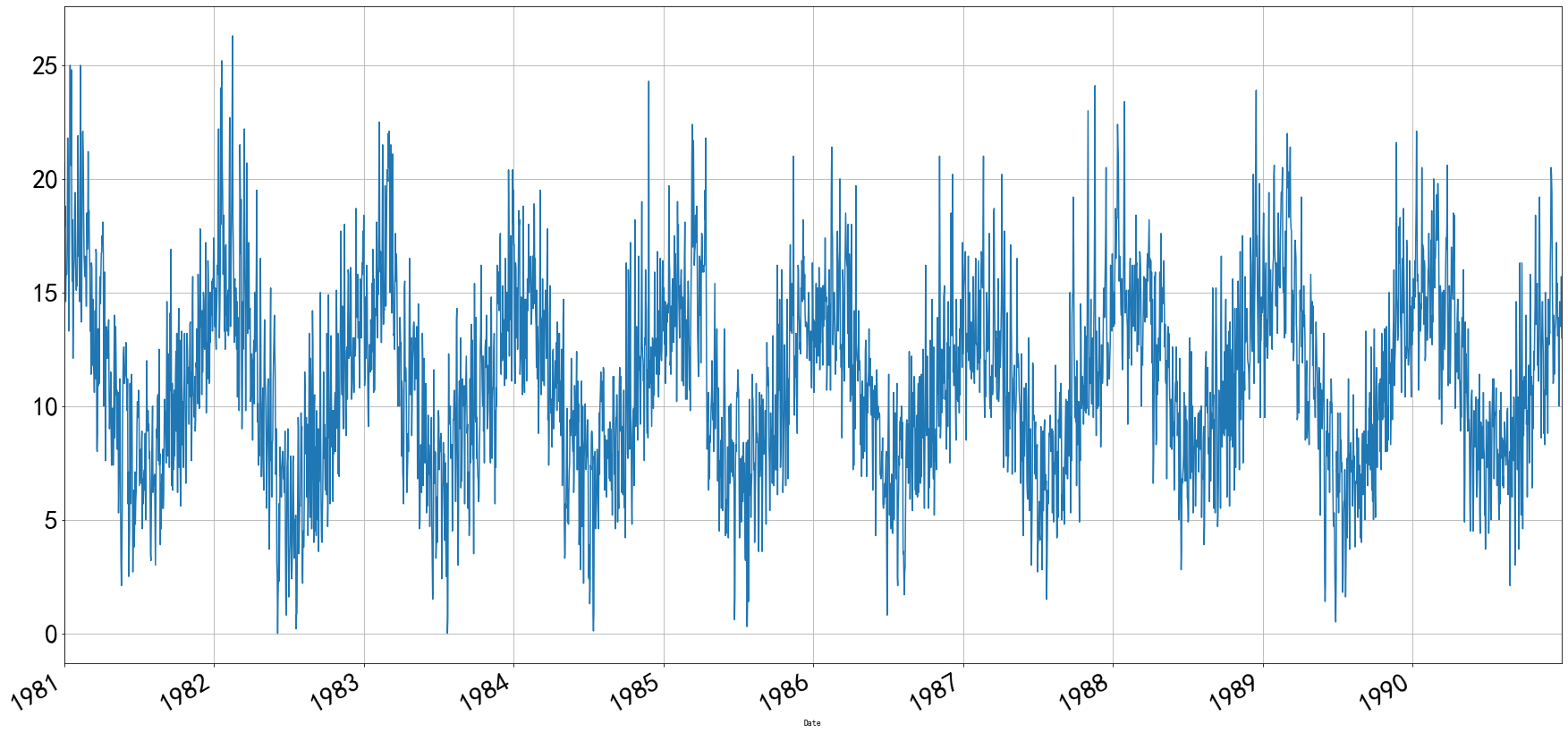3.3.2 散点图
"""绘制散点图"""
df['Temp'].plot(style='k.', figsize=(30,15))
# 设置坐标字体大小
plt.tick_params(labelsize=30)
# 生成刻度线网格
plt.grid()

结果：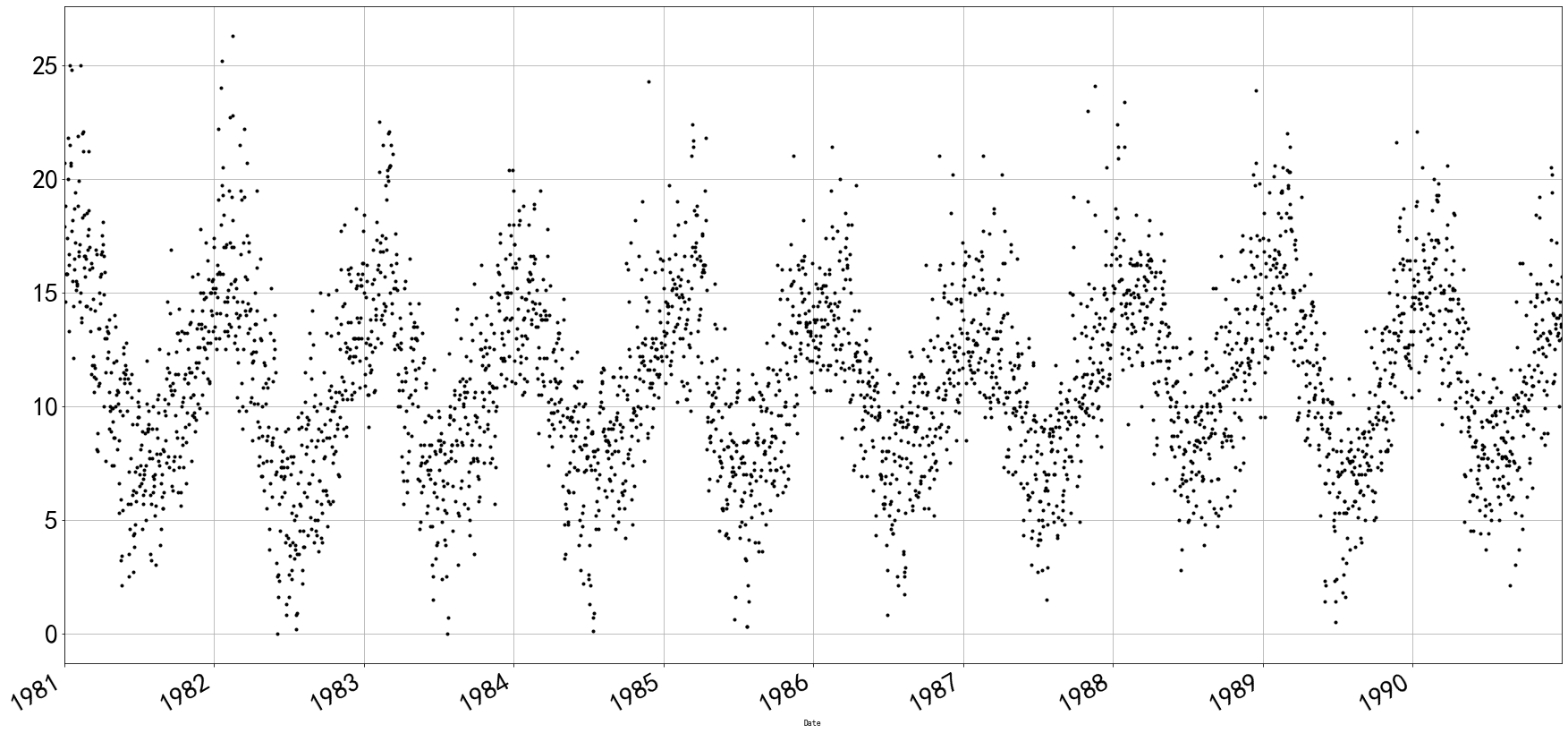3.3.3 直方图
# 设置绘图大小
plt.style.use({'figure.figsize':(5, 5)})
df['Temp'].plot(kind='hist', bins=20)  # bins参数表示横轴多少等分

# 还有一种
df['Temp'].hist(bins=50)

结果：3.3.4 堆积面积图
# 设置绘图大小
plt.style.use({'figure.figsize':(10, 5)})
df.plot.area(stacked=False)

结果：3.3.5 核密度估计图（KDE图）
# 设置绘图大小
plt.style.use({'figure.figsize':(10, 5)})
df['Temp'].plot(kind='kde')

结果：3.3.6 热力图
将1982年每一个月的最低气温用热力图展示出来 关于pandas的重采样resample
# 设置绘图大小
plt.style.use({'figure.figsize':(20, 8)})

df['1982'].resample('M').mean().T

sns.heatmap(df['1982'].resample('M').mean().T)

"""为什么6月和7月气温最低？  因为墨尔本在澳大利亚，属于南半球，6月和7月是冬天"""

结果：3.4 绘制每年气温变化的直线图，箱型图，热力图，小提琴图
我们刚刚将日期列转换成了pandas中的datetime类型，我们可以直接通过年份和日期索引选择指定时间的数据
df['1984']

下面实用pandas的groupby操作，把每年和每天的温度筛选出来python处理数据的风骚操作pandas 之 groupby&agg
groups = df.groupby(pd.Grouper(freq='1Y'))['Temp']
years = pd.DataFrame()
for name, group in groups:
years[name.year] = group.values

years

这一个要看一下实现了什么效果：这个就是把十年的每一天的数据都给做了出来，每一列代表年，每一行是天。下面绘制图像进行可视化上面这个表：
# 设置绘图大小
plt.style.use({'figure.figsize':(30, 15)})

years.plot()

# 设置图例文字大小和图示大小
plt.legend(fontsize=15, markerscale=15)
# 设置坐标文字大小
plt.tick_params(labelsize=15)

结果：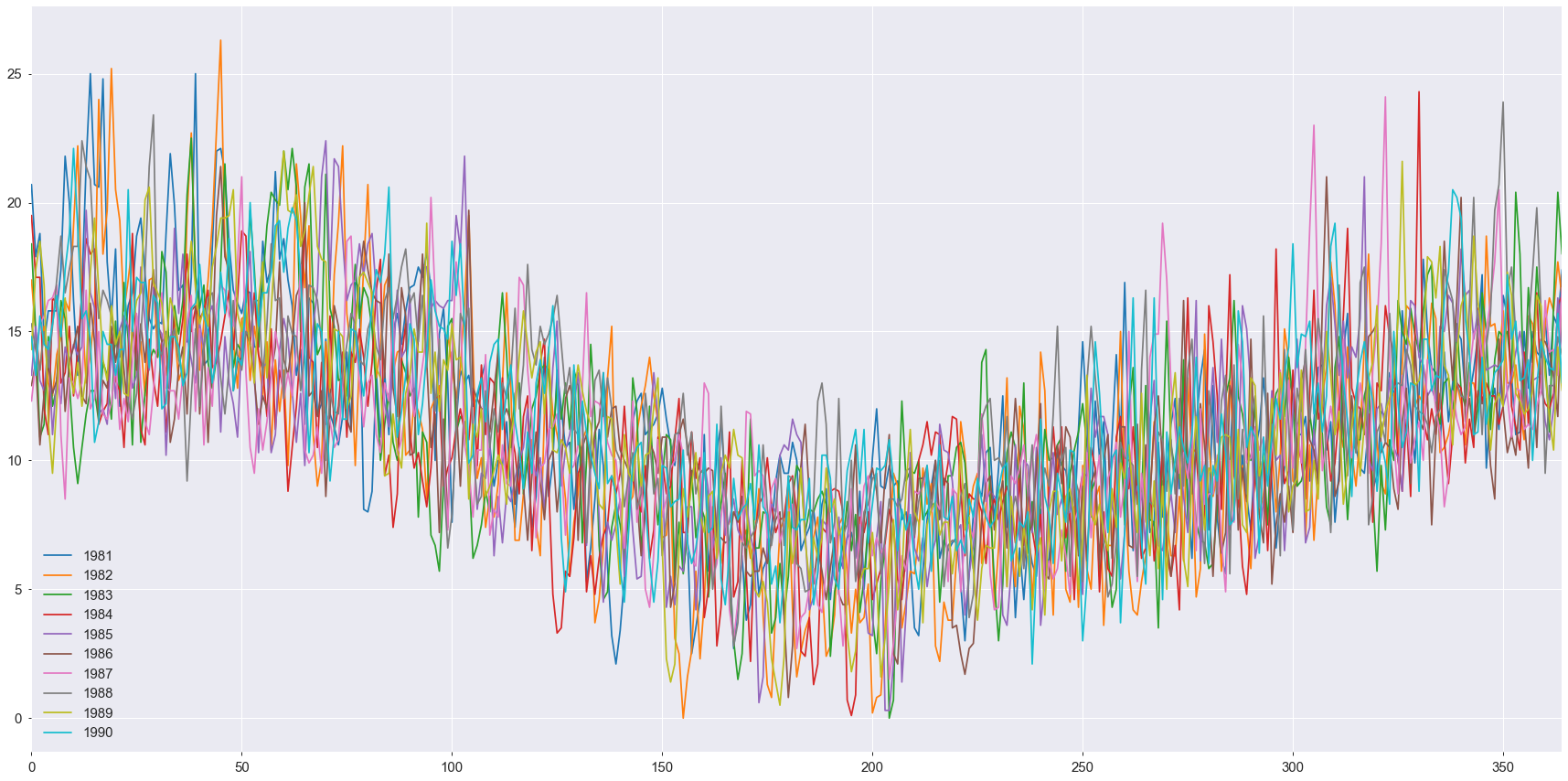3.4.1 折线图
"""折线图"""
years.plot(subplots=True, figsize=(20, 45))
plt.show()

这个会绘制每一年的折线图，结果就不全显示了：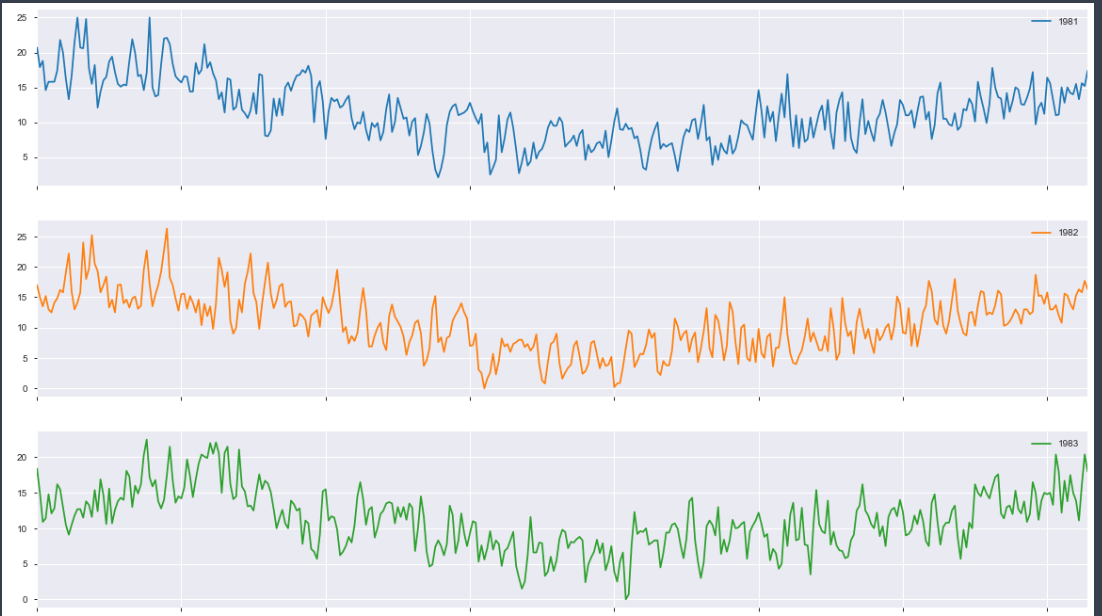3.4.2 箱型图
这个图可以很容易的看出离群点和数据的分布
"""箱型图"""
years.boxplot(figsize=(20, 10))

结果如下：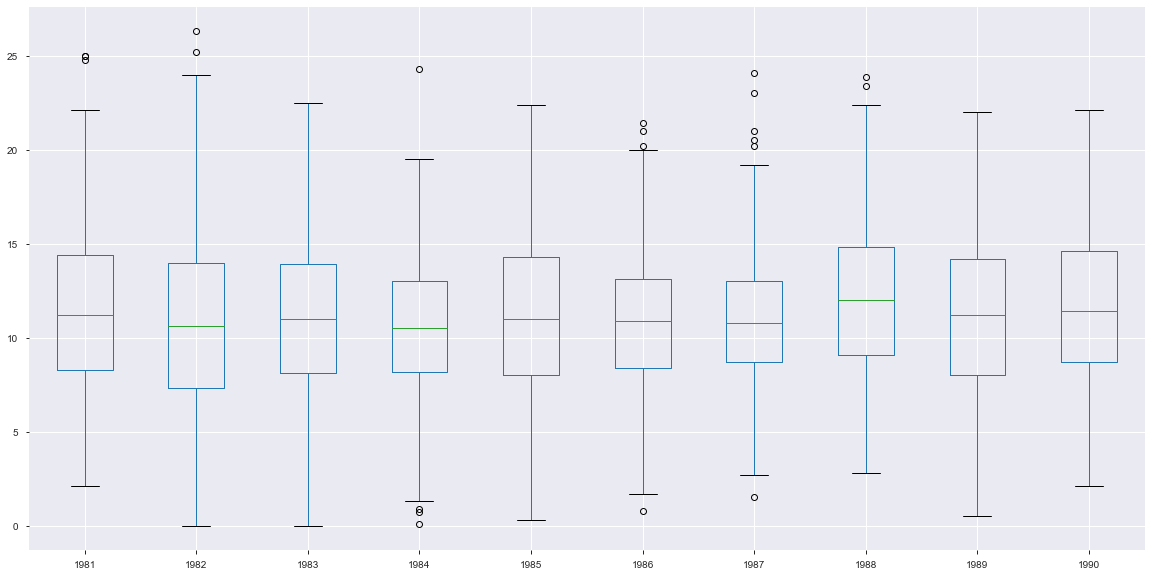3.4.3 热力图
# 设置绘图大小
plt.style.use({'figure.figsize':(30, 10)})
sns.heatmap(years.T)
"""颜色越黑，表示温度越低， 颜色越亮，表示温度越高"""

结果：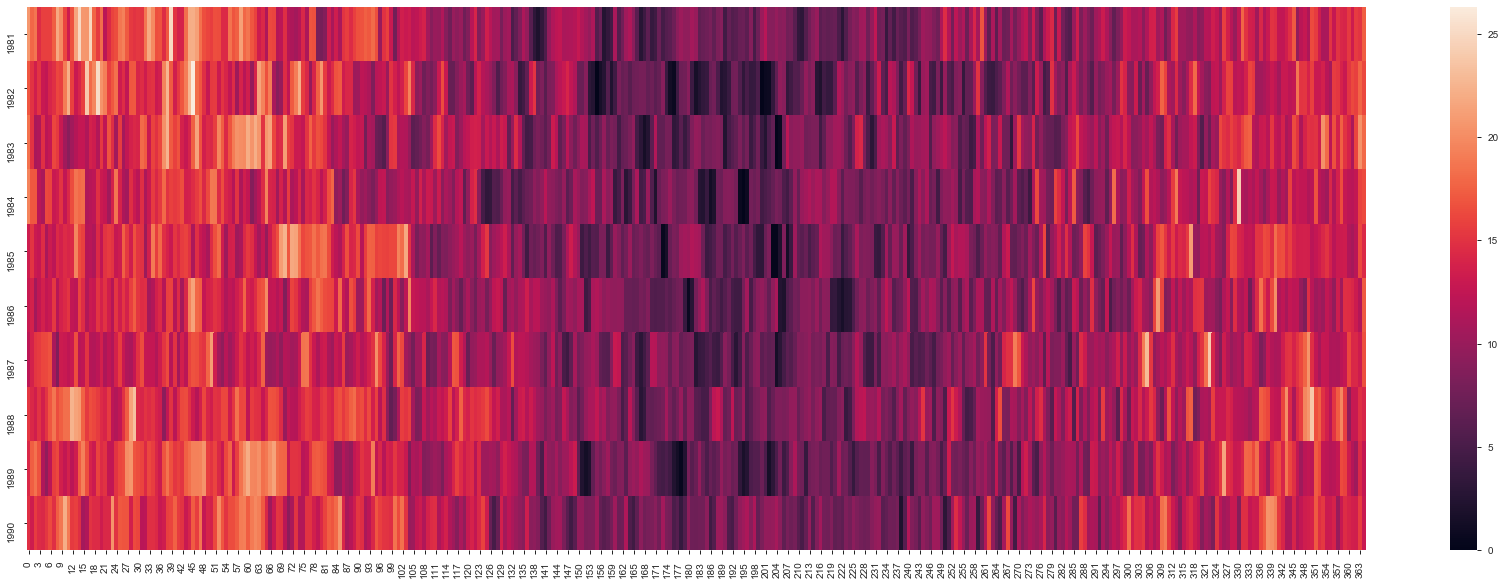plt.matshow(years.T, interpolation=None, aspect='auto')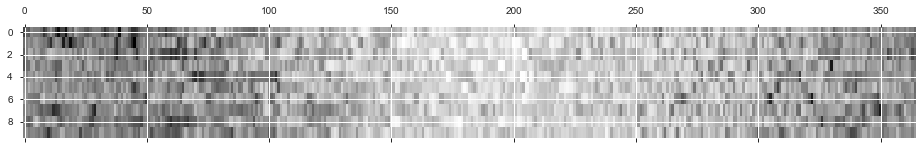3.4.4 每一年气温的直方图
"""每一年气温的直方图"""
plt.style.use({'figure.figsize':(30, 22)})
years.hist(bins=15)

这个不做展示。
下面绘制1985年12个月每天的气温数据，这个和上面的异曲同工，只不过那个是每一年的每一天，这个是每一个月的每一天。
# 选取1985年12个月每天的气温数据
groups_month = df['1985'].groupby(pd.Grouper(freq='1M'))['Temp']

months = pd.concat([pd.DataFrame(x.values) for x in groups_month], axis=1)
months = pd.DataFrame(months)
months.columns = range(1, 13)
months

看一下months：绘制箱型图：
months.boxplot(figsize=(20, 15))
plt.title('墨尔本1982年每个月最低温度分布箱型图')

绘制热力图：
# 设置图像大小
plt.style.use({'figure.figsize':(5, 8)})
sns.heatmap(months)
plt.title('墨尔本1982年每天最低气温分布热力图')

plt.matshow(months, interpolation=None, aspect='auto', cmap='rainbow')

3.4.5 小提琴图
类似箱型图，只不过比箱型图更加高级一些
# 设置图像大小
plt.style.use({'figure.figsize':(15, 10)})

sns.violinplot(data=months)
plt.title('墨尔本1982年每个月最低气温分布小提琴图')

# linewidth参数可以控制线宽
sns.violinplot(data=months, linewidth=3)
plt.title('墨尔本1982年每个月最低气温分布小提琴图')

结果：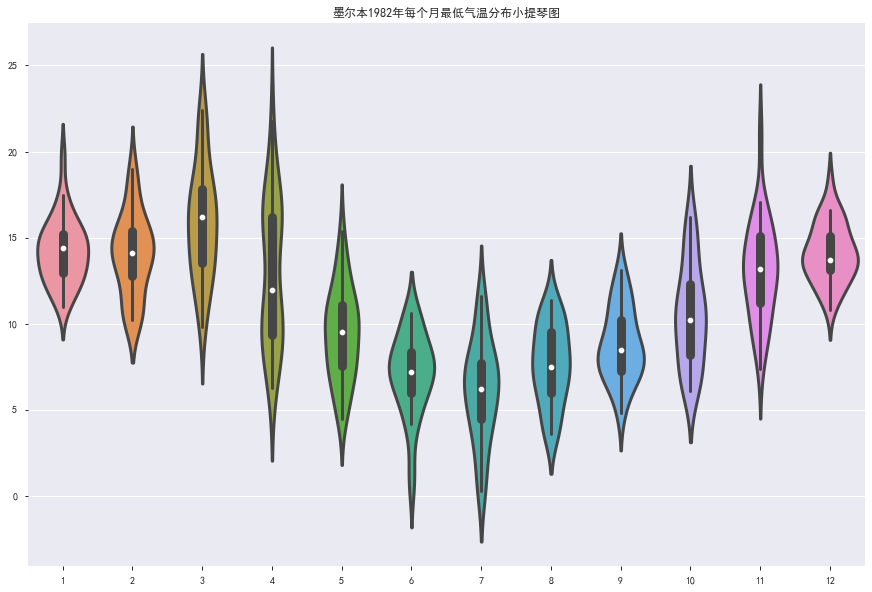3.5 滞后散点图
时间序列分析假定一个观测值与前面的观测值之间存在一定的关系。 相对于某观察值之前的观测值被称为滞后值，在一个时间步长前的观测值称为滞后一期，在两个时间步长前的观测值称为滞后二期，依次类推。 比如， 对于1982年8月15日的气温数据，8月14日的气温为滞后一期，8月13日的气温为滞后二期 每个观察值之间和其滞后值之间的关系，可以用滞后散点图表示
from pandas.plotting import lag_plot

# 设置图像大小
plt.style.use({'figure.figsize':(10, 10)})

lag_plot(df['Temp'])
plt.title('墨尔本1980-1990年最低气温滞后1期散点图')

结果：散点图聚在左下角到右上角，表示与滞后值正相关。 散点图聚在左上角到右下角，表示与滞后值负相关。 离对角线越紧密，表示相关关系越强。 分散的球状散点图表示相关关系微弱。
横轴表示每天的气温，纵轴表示滞后一天的气温
通过lag参数控制滞后值
lag_plot(df['Temp'], lag=3)
plt.title('墨尔本1980-1990年最低气温滞后3期散点图')

结果：还可以绘制不同滞后值对应的散点图：
"""绘制不同滞后值对应的滞后散点图"""
lag_list = [1, 2, 3, 5, 10, 20, 50, 100, 150, 180]
plt.style.use({'figure.figsize':(15, 35)})
for i in range(len(lag_list)):
ax = plt.subplot(5, 2, i+1)
ax.set_title('t vs t+' + str(lag_list[i]))
lag_plot(df['Temp'], lag=lag_list[i])
plt.title('墨尔本1980-1990年最低气温滞后{}期散点图'.format(lag_list[i]))

3.6 自相关图
这个图还是比较重要的，尤其是对于时间序列来说，可以看出周期和相关性。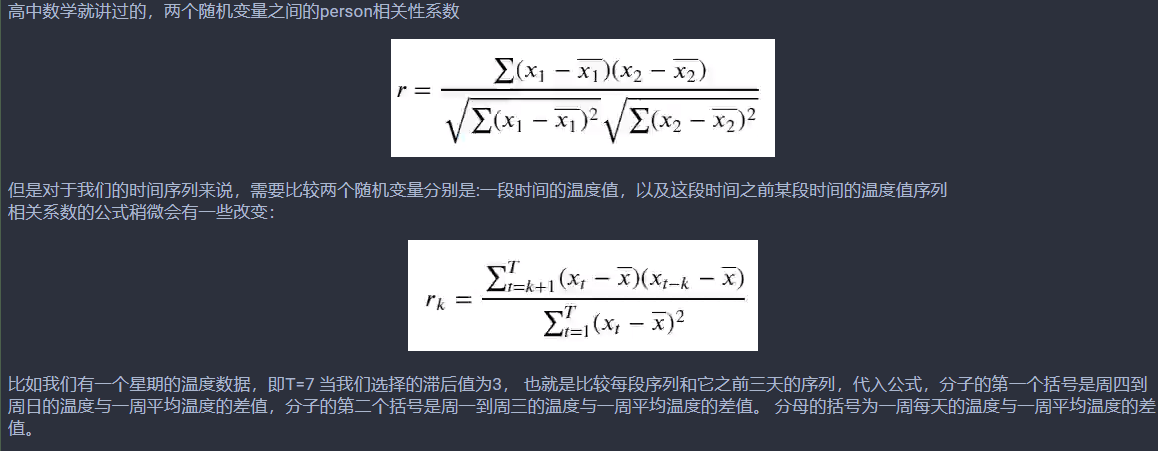代码如下：
from pandas.plotting import autocorrelation_plot

# 设置图像大小
plt.style.use({'figure.figsize':(15, 10)})

autocorrelation_plot(df['Temp'])
plt.title('墨尔本1980-1990最低气温自相关图')

# 设置坐标文字大小
plt.tick_params(labelsize=10)
plt.yticks(np.linspace(-1, 1, 120))

结果如下：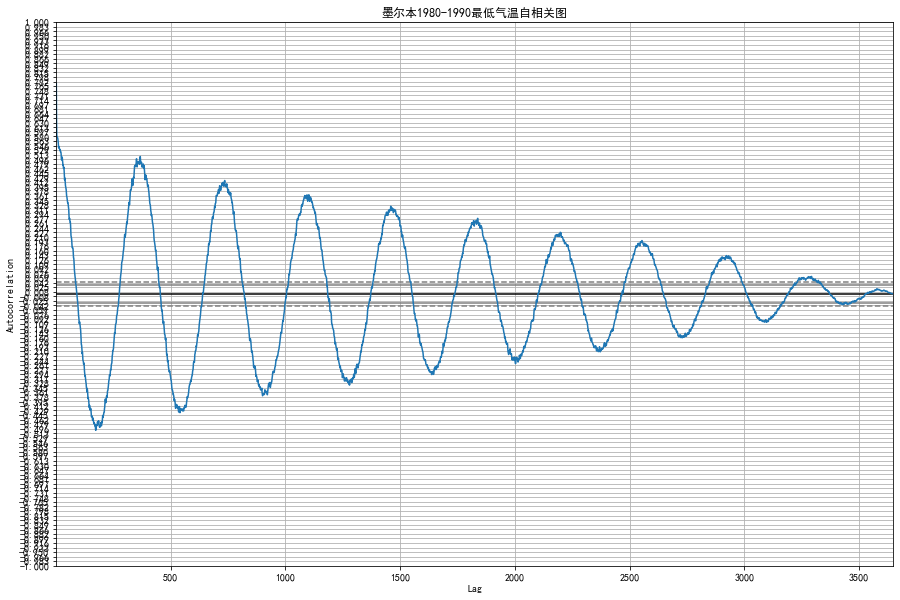比如说横轴是1000， 就表示这个数据集所有数据与它之后1000天的数据的自相关程度。
时间间隔的越远，温度之间的自相关程度就摆动着降低。 并且在时间向远推进的过程中，还会出现周期性的波动，这是由季节的周期性更迭造成的
如果我们随机取点绘制滞后图和自相关图，就会发现完全没有规律。
# 设置图像大小
plt.style.use({'figure.figsize':(10, 5)})

a = np.random.randn(100)
a = pd.Series(a)
lag_plot(a)
plt.title("随机数列的1期滞后散点图")

结果：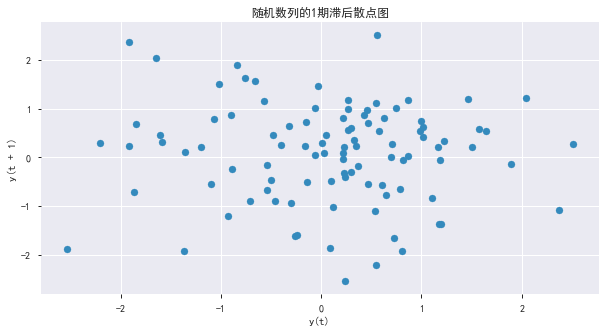autocorrelation_plot(a)
plt.title('随机数列的自相关图')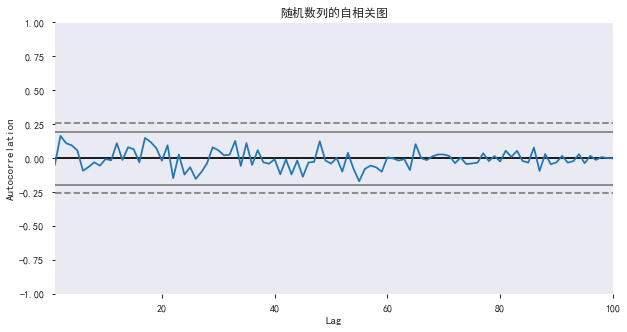4. 时间数据的特征工程
将单纯的日期维度扩展成更多维度，构造更多的输入给模型的特征，增强数据的可解释性，更接近人的业务逻辑。
哪一年哪一个月星期几这个月的第几天月初还是月末一年当中的第几天一年当中的第几个月
# 重新调用pandas的read_csv函数读取数据集文件
df2['Date'] = pd.to_datetime(df2['Date'])

下面开始构造数据特征：
把年月日作为特征构造成列 # 构造新的一列： 年
df2.loc[:, 'year'] = df2['Date'].apply(lambda x: x.year)

# 构造新的一列： 月
df2.loc[:, 'month'] = df2['Date'].apply(lambda x: x.month)

# 构造新的一列： 星期几
df2.loc[:, 'dow'] = df2['Date'].apply(lambda x:x.dayofweek)

# 构造新的一列： 一个月第几天
df2.loc[:, 'dom'] = df2['Date'].apply(lambda x: x.day)
  是不是周末，是不是周六，是不是周日 # 构造新的三列： 是不是周末、是不是周六、是不是周日
df2.loc[:, 'weekend'] = df2['Date'].apply(lambda x:x.dayofweek > 4)
df2.loc[:, 'weekend_sat'] = df2['Date'].apply(lambda x: x.dayofweek == 5)
df2.loc[:, 'weekend_sun'] = df2['Date'].apply(lambda x: x.dayofweek == 6)
  添加上半月和下半月的信息
# 添加上半月和下半月的信息
def half_month(day):
if day in range(1, 16):
return 1
else:
return 2

df2.loc[:, 'half_month'] = df2['dom'].apply(lambda x:half_month(x))

添加每个月上中下旬的信息
# 添加每个月上中下旬的信息
def three_part_month(day):
if day in range(1, 11):
return 1
if day in range(11, 21):
return 2
else:
return 3

df2.loc[:, 'three_part_month'] = df2['dom'].apply(lambda x: three_part_month(x))

添加每个月四个星期的信息 一个月的第几个星期
# 添加每个月四个星期的信息  一个月的第几个星期
def four_week_month(day):
if day in range(1, 8):
return 1
if day in range(8, 15):
return 2
if day in range(15, 22):
return 3
else:
return 4

df2.loc[:, 'four_week_month'] = df2['dom'].apply(lambda x: four_week_month(x))

添加节假日信息
# 添加节假日信息
df2.loc[:, 'festival'] = 0
df2.loc[(df2.month==1) & (df2.dom<4), 'festival'] = 1

看看结果，已经从原来的一列气温出来了好多个时间特征，这些对于后面模型会有用。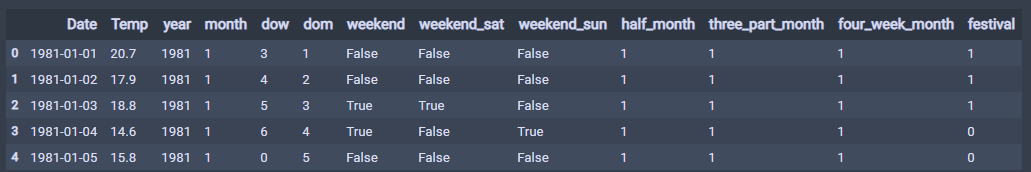有了好的特征才有好的模型的输入，并且对于一年的季度还有一个月的上中下旬，这样用1234表示的定类数据，计算机并不能理解什么意思，123表示上中下，并不意味着下旬就比上旬大2，所以需要转成独热编码的形式显示。
5. 独热编码One-Hot Encoding
独热编码用来将定类数据表示成0-1二进制，便于输入模型中。
比如，计算机并不认识颜色这一列的“红”， “黄”， “绿”三个分类，所以我们用“是不是红色”， “是不是黄色”， “是不是绿色”三列来分开“颜色”这一列特征。
下面开始做独热编码，但是在做独热编码之前，先保存一份数据
before_dummy_df = df2.copy()

# 构造数据集的特征
drop_columns = ['Date', 'Temp']
X_before_dummy = before_dummy_df.drop(drop_columns, axis=1)

# 构造数据集的标签
Y = df['Temp']

"独热向量编码"
columns_to_encoding = ['year', 'month', 'dow', 'dom', 'three_part_month', 'four_week_month']

# 使用pandas的get_dummyise函数对df2指定的列进行独热向量编码
dummy_X = pd.get_dummies(X_before_dummy, columns=columns_to_encoding)

独热编码之后，就会发现数据有了72列特征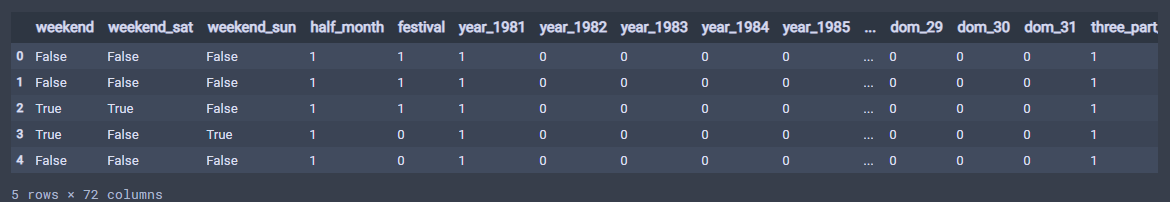我们通过上面，也构造出了X和Y了，到现在我们有两组数据：
没有经过独热向量编码操作的数据X_befor_dummy经过独热向量编码的数据dummy_X
共有标签Y。
有了数据，我们就可以建立模型进行拟合了。
6. 机器学习模型的回归拟合
准备好了数据集，就可以进行回归拟合了，我们先用几个常见的解决回归问题的机器学习模型：
多元线性回归多项式回归岭回归决策树和随机森林支持向量机多层感知机（多层神经网络）
回归模型的评估指标：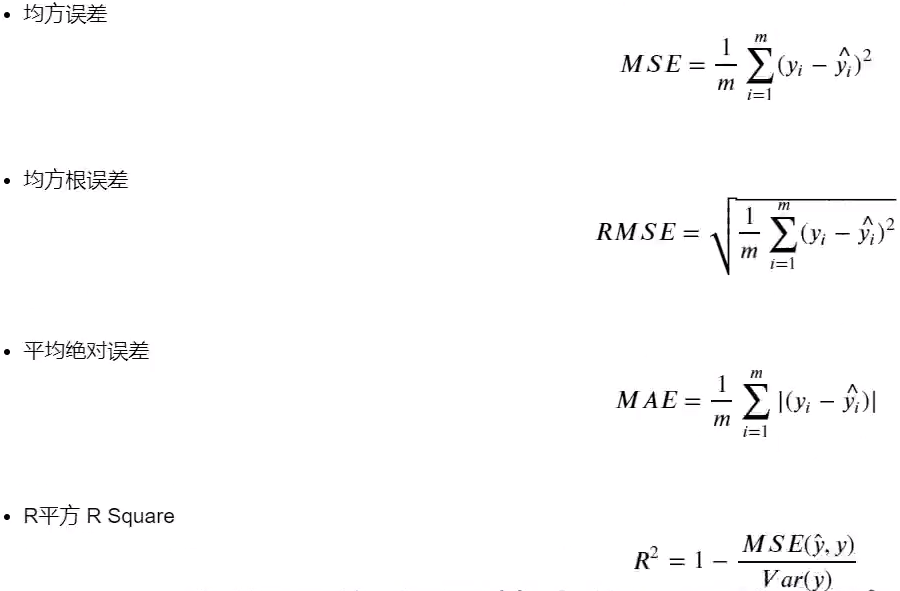6.1 多元线性回归拟合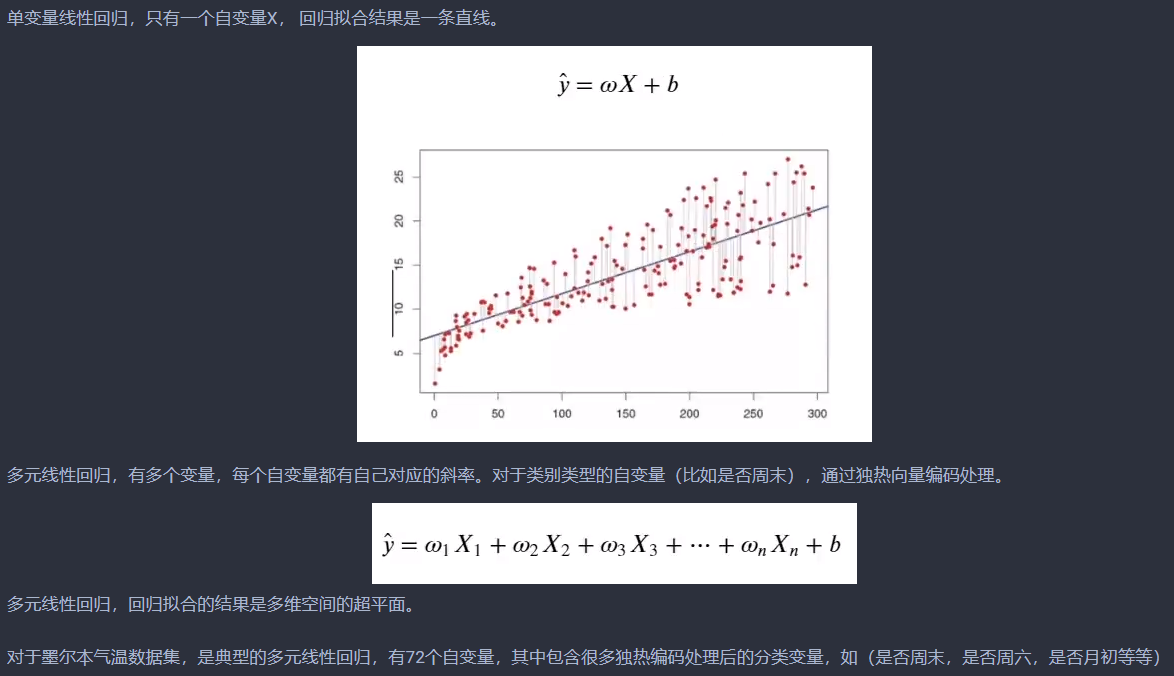代码如下：
from sklearn.model_selection import train_test_split
from sklearn.linear_model import LinearRegression

# 划分训练集和验证集
x_train, x_test, y_train, y_test = train_test_split(dummy_X, Y, test_size=0.2, random_state=1, shuffle=True)

lr_reg = LinearRegression()
lr_reg.fit(x_train, y_train)
print('截距', lr_reg.intercept_)
print('斜率（线性模型中各特征对应的系数）', lr_reg.coef_)

下面把拟合效果进行可视化：
# 绘制气温散点图
df['Temp'].plot(style='k.', figsize=(20, 10))
df.loc[:, '线性回归'] = lr_reg.predict(dummy_X)
plt.plot(df['线性回归'], 'r.')

# 设置坐标文字大小
plt.tick_params(labelsize=20)

# 生成刻度线网格
plt.grid()

结果如下：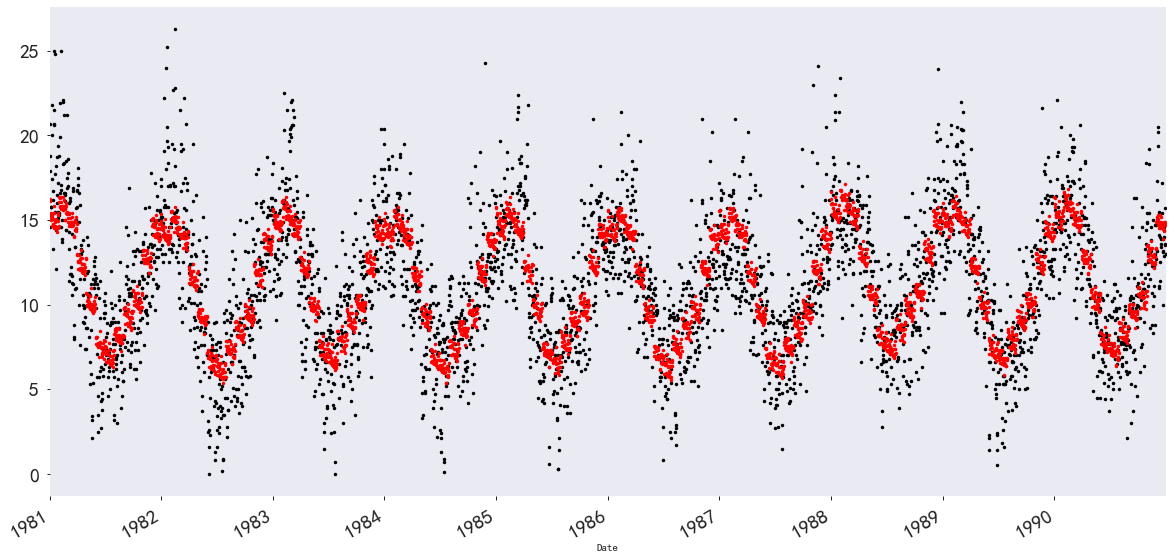6.2 二项多项式回归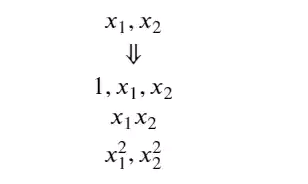代码如下：
from sklearn.preprocessing import PolynomialFeatures

# 构建一个特征处理器poly_reg, 它能将输入特征变成二次的，见上面图
poly_reg = PolynomialFeatures(degree=2)

# 使用构建的二项多项式特征处理器poly_reg处理训练数据dummy_X, 得到包含二次特征的训练集X_poly
X_poly = poly_reg.fit_transform(dummy_X)

解释一下上面的代码：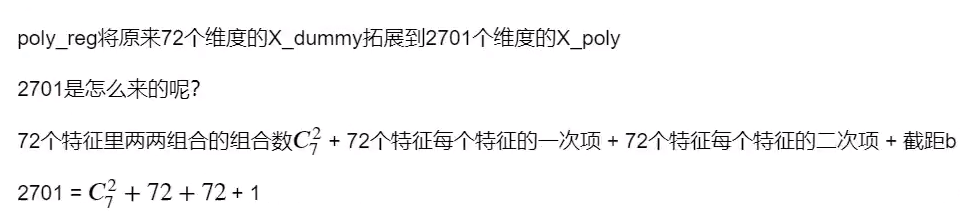构建二次多项式回归模型，将上面构造好的X_poly送进去训练
lin_reg_2 = LinearRegression()
lin_reg_2.fit(X_poly, Y)

# 查看回归方程系数
print('每个维度对应的系数（斜率）：Cofficients: ', lin_reg_2.coef_)

# 查看回归方程截距
print('截距： intercept', lin_reg_2.intercept_)
df.loc[:, '二次多项式回归'] = lin_reg_2.predict(X_poly)

可视化结果：
# 绘制二次多项式回归模型拟合的气温
df.loc[:, '二次多项式回归'] = lin_reg_2.predict(X_poly)
plt.plot(df['二次多项式回归'], 'g*')

# 绘制气温散点图
df['Temp'].plot(style='k.', figsize=(20, 13))

# 设置图例文字大小和图示大小
plt.legend(fontsize=25, markerscale=5)

# 设置坐标文字大小
plt.tick_params(labelsize=25)

# 生成网格
plt.grid()

结果如下：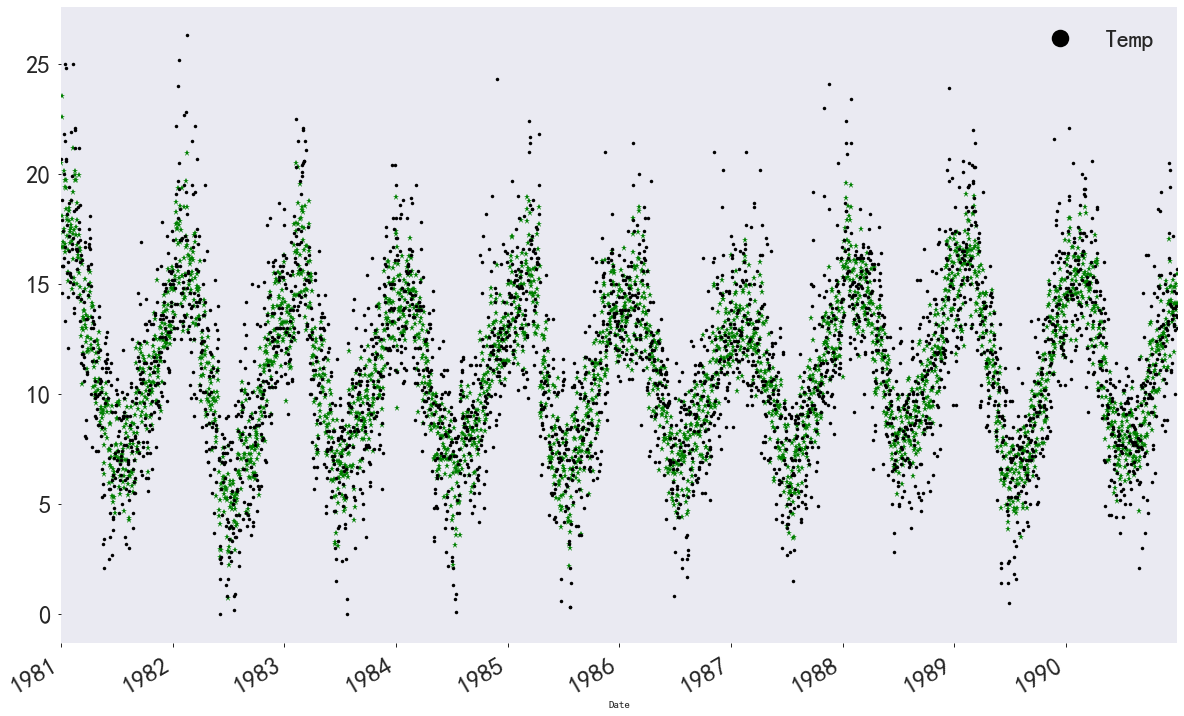三次多项式回归模型：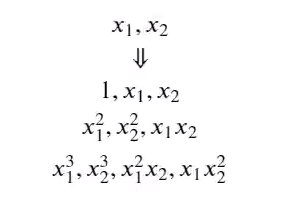# 构建一个特征处理器ploy_reg3, 它能将输入特征变成三次的
ploy_reg3 = PolynomialFeatures(degree=3)

# 使用构建的三次多项式处理训练集dummy_X， 得到包含三次特征的训练集X_poly3
X_poly3 = ploy_reg3.fit_transform(dummy_X)

# 构建三次多项式回归模型并训练
lin_reg_3 = LinearRegression()
lin_reg_3.fit(X_poly3, Y)
df.loc[:, '三次多项式回归'] = lin_reg_3.predict(X_poly3)

这个可视化一下，就会发现这个会过拟合：
# 绘制二次多项式回归模型拟合的气温, 绿色五角星表示
plt.plot(df['二次多项式回归'], 'g*')
# 绘制三次多项式回归模型拟合的气温，红色三角形表示
plt.plot(df['三次多项式回归'], 'r^')

# 绘制气温散点图， 黑色点表示
df['Temp'].plot(style='k.', figsize=(20, 13))

# 设置图例文字大小和图示大小
plt.legend(fontsize=15, markerscale=3)

# 设置坐标文字大小
plt.tick_params(labelsize=25)

# 生成网格
plt.grid()

"""这个三项式的这个明显出现了过拟合现象"""

结果：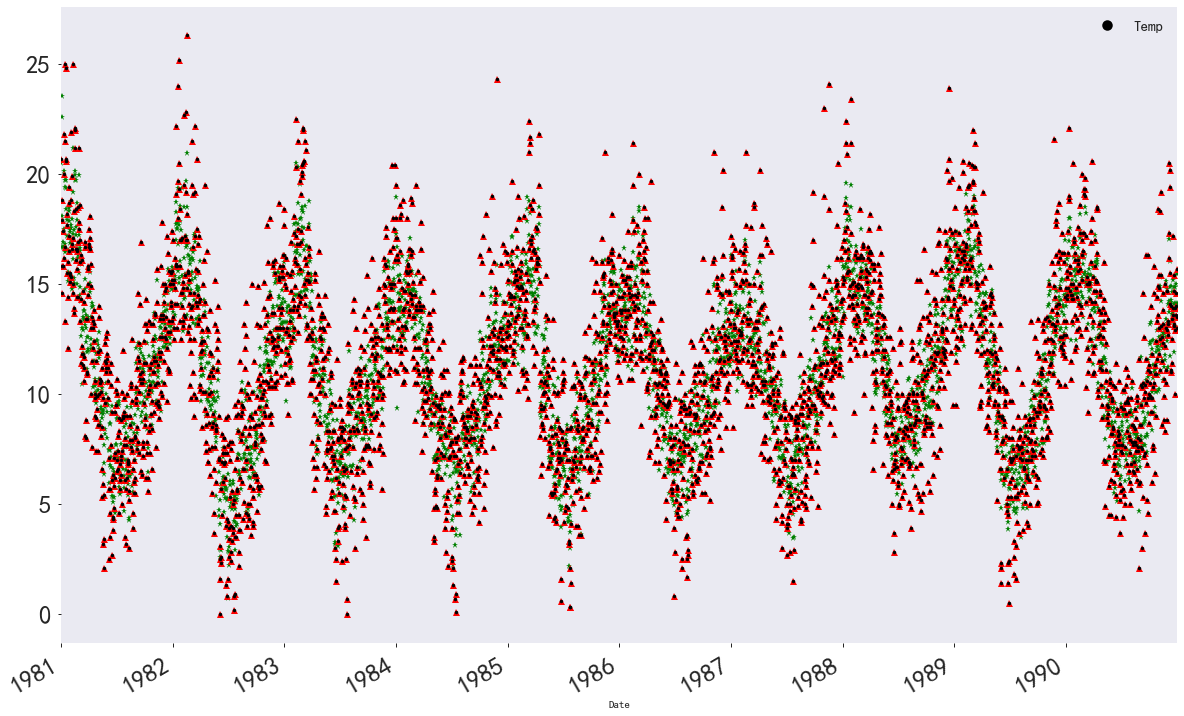三次多项式拟合的点与原始数据完全重合，出现了过拟合。 在机器学习中，我们希望模型能大而化之的学习到数据普遍一般规律，而不是对每一个点死记硬背。 因此，线性回归中，二项多项式回归效果是最好的。
6.3 岭回归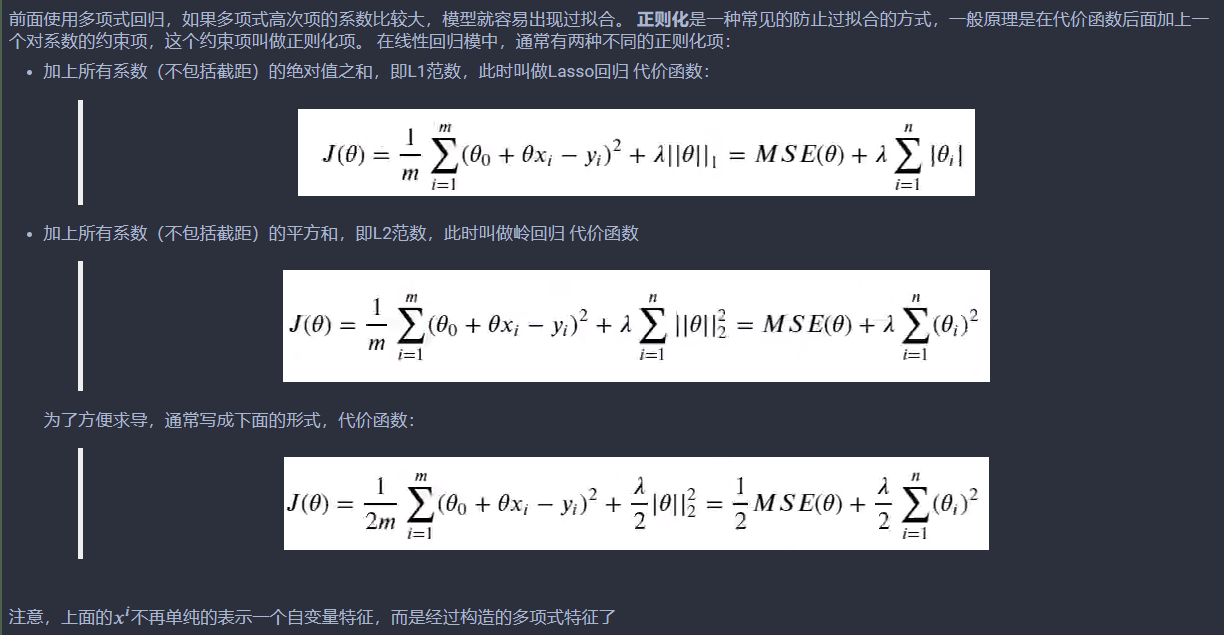代码如下：
from sklearn.model_selection import train_test_split
x_train, x_test, y_train, y_test = train_test_split(dummy_X, Y, test_size=0.2, random_state=1, shuffle=True)

from sklearn.linear_model import RidgeCV

# alphas 表示正则化强度， 类似之前的代价函数中的λ， cv=5表示5折交叉验证
ridge = RidgeCV(alphas=[0.2, 0.5, 0.8], cv=5)
ridge.fit(x_train, y_train)

ridge.score(x_test, y_test)

可视化一下：
# 绘制气温散点图， 用黑色点表示
df['Temp'].plot(style='k.', figsize=(20, 13))

df.loc[:, '岭回归'] = ridge.predict(dummy_X)
plt.plot(df['岭回归'], 'g.')

# 设置图例文字大小和图示大小
plt.legend(fontsize=15, markerscale=3)

# 设置坐标文字大小
plt.tick_params(labelsize=25)

# 生成刻度线网格
plt.grid()

结果：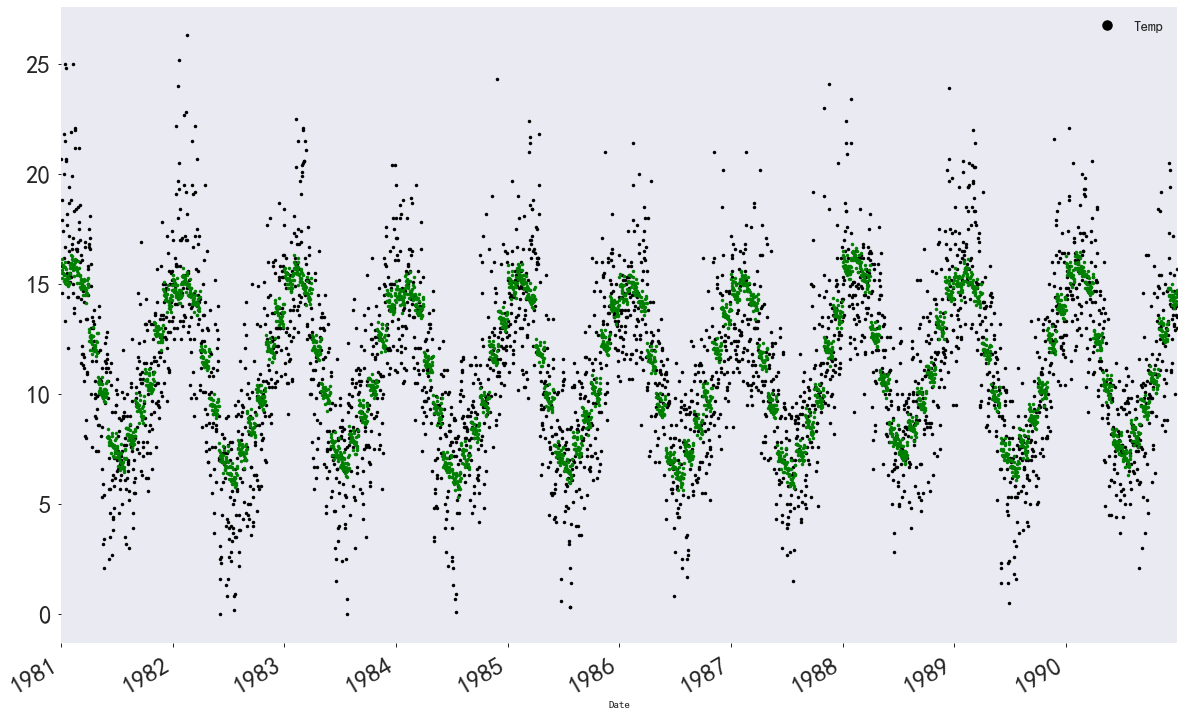6.4 决策树和随机森林
决策树和随机森林的训练数据不需要进行独热向量编码，所以我们可以直接用X_before_dummy。
"""划分训练集和测试集"""
x_train, x_test, y_train, y_test = train_test_split(X_before_dummy, Y, test_size=0.2, random_state=1, shuffle=True)

# 从Python机器学习与数据挖掘工具库sklearn导入随机森林回归器
from sklearn.ensemble import RandomForestRegressor

# 导入网格搜索交叉验证，网格搜索可以让模型参数按我们给定的列表遍历，找到效果最好的模型
# 交叉验证可以充分评估模型的准确性
from sklearn.model_selection import GridSearchCV

# 构造参数字典
param_grid = {
'n_estimators': [5, 10, 20, 50, 100, 200],    # 决策树的个数
'max_depth': [3, 5, 7],   # 最大树身， 树太深会造成过拟合
'max_features': [0.6, 0.7, 0.8, 1]  # 决策树划分时考虑的最大特征数
}

rf = RandomForestRegressor()

# 以随机森林回归器为基础构造网格搜索回归器
grid = GridSearchCV(rf, param_grid=param_grid, cv=3)

# 在训练集上训练
grid.fit(x_train, y_train)

"""选取最优参数对应的模型"""
# 查看效果最好的参数
grid.best_params_
# 指定模型效果最好的参数对应的模型
rf_reg = grid.best_estimator_

# 可视化决策树
from sklearn import tree
import pydotplus
from IPython.display import Image, display

# 从随机森立中选取一棵决策树进行可视化
estimator = rf_reg.estimators_

dot_data = tree.export_graphviz(estimator, out_file=None, filled=True, rounded=True)
graph = pydotplus.graph_from_dot_data(dot_data)
display(Image(graph.create_png()))

6.4.1 特征重要度分析
"""特征重要度分析"""
rf_reg.feature_importances_

print('特征排序')

feature_names = ['year', 'month', 'dow', 'dom', 'weekend', 'weekend_sat', 'weedend_sun', 'half_month',
'three_part_month', 'four_week_month', 'festival']

feature_importances = rf_reg.feature_importances_
indics = np.argsort(feature_importances)[::-1]

for index in indics:
print('features %s (%f)' %(feature_names[index], feature_importances[index]))
plt.figure(figsize=(16, 8))

plt.title('随机森立模型不同特征的重要度')
plt.bar(range(len(feature_importances)), feature_importances[indics], color='b')
plt.xticks(range(len(feature_importances)), np.array(feature_names)[indics], color='b')

可以看到特征重要程度：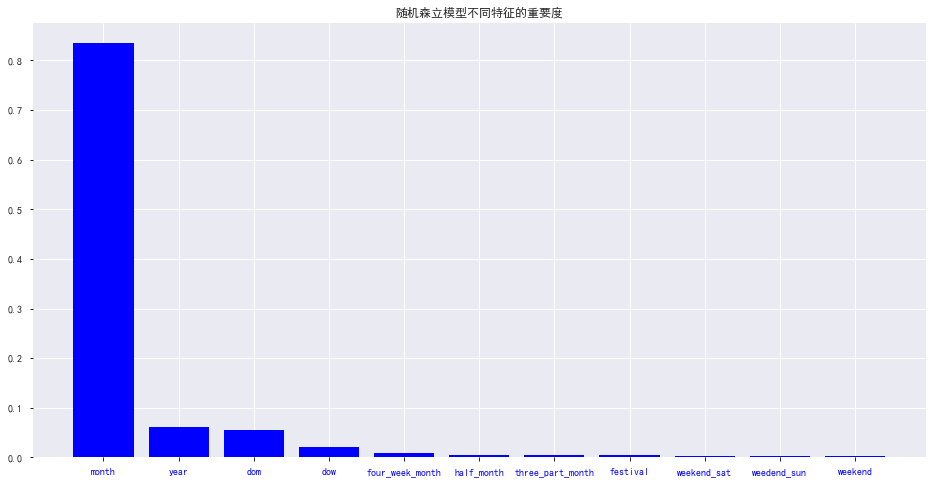6.4.2 可视化回归拟合效果
# 绘制气温散点图
df['Temp'].plot(style='k.', figsize=(20, 15))

# 绘制随机森林模型拟合的气温
df.loc[:, '随机森林'] = rf_reg.predict(X_before_dummy)
plt.plot(df['随机森林'], 'r.')

# 绘制岭回归模型拟合的气温
plt.plot(df['岭回归'], 'g.')

plt.legend(fontsize=15, markerscale=3)

plt.tick_params(labelsize=25)

plt.grid()

结果：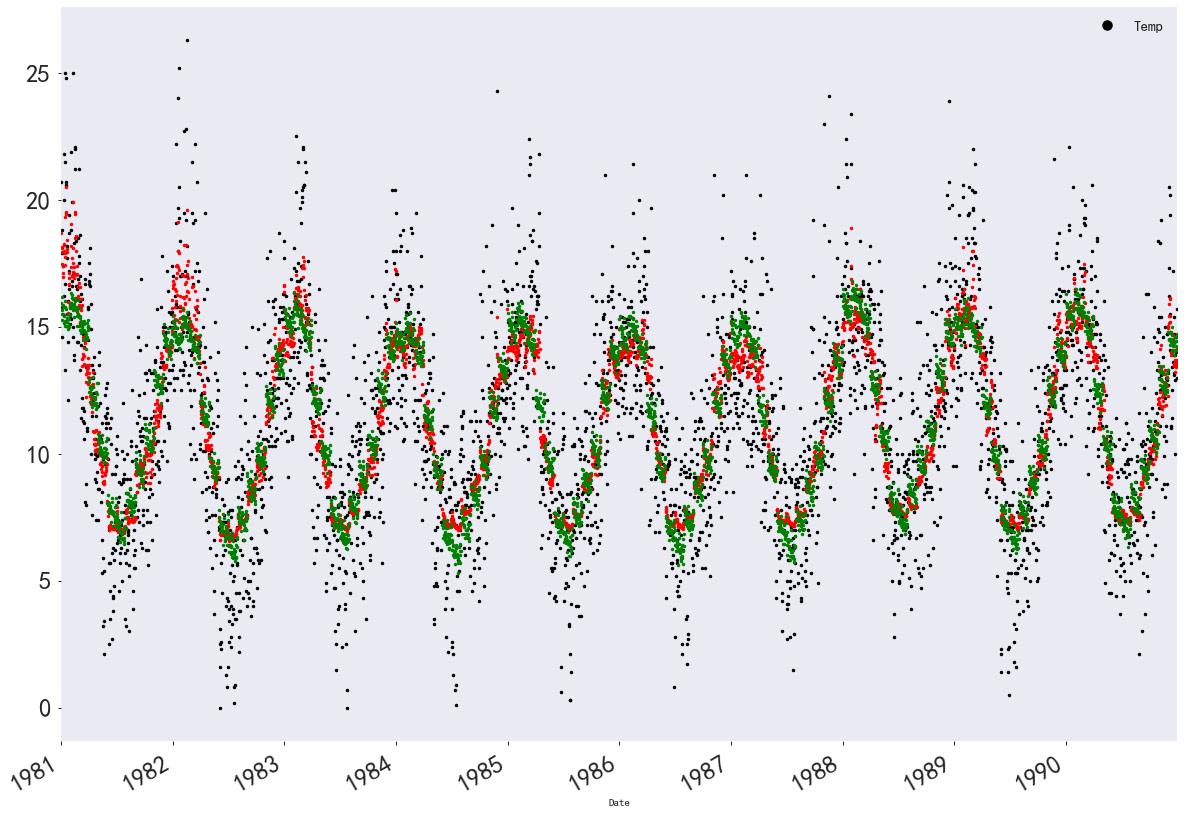6.5 多层神经网络
神经网络对输入特征的幅度很敏感，我们首先需要将输入特征归一化
from sklearn.preprocessing import scale

feature = scale(X_before_dummy)
X_train, X_val, Y_train, Y_val = train_test_split(feature, Y, test_size=0.2, random_state=1, shuffle=True)

"""训练集共有2920条数据， 对应2920天的11个时间特征，标签为对应的气温"""
# 使用Keras模型快速搭建全连接神经网络
from keras.models import Sequential
from keras.layers.core import Dense, Dropout
from keras.optimizers import SGD

model = Sequential()

# 第一层，32个神经元，激活函数是relu， 接收11个特征作为输入

# 第二层，64个神经元，激活函数为relu

# 回归模型的神经网络最后一层不需要有激活函数，直接用一个神经元线性输出结果即可

# 将模型封装好，使用均方误差mse作为损失函数，使用学习率0.001的随机梯度下降算法反向传播，同时平均绝对误差mae监控模型训练效果
model.compile(loss='mse', optimizer=SGD(lr=0.001), metrics=['mae'])

# 训练神经网络, 每一批128个数据， 训练50轮（即过50遍完整的训练集）， 每一轮结束后用验证集评估模型效果
network_history = model.fit(X_train, Y_train, batch_size=128, epochs=50, verbose=1, validation_data=(X_val, Y_val))

绘制结果误差：
"""绘制训练过程的mse误差和mae误差"""
def plot_history(network_history):
plt.figure()
plt.xlabel('Epochs')
plt.ylabel('Loss')
plt.plot(network_history.history['loss'])
plt.plot(network_history.history['val_loss'])
plt.legend(['Training', 'Validation'])

plt.figure()
plt.xlabel('Epochs')
plt.ylabel('Mae')
plt.plot(network_history.history['mean_absolute_error'])
plt.plot(network_history.history['val_mean_absolute_error'])
plt.legend(['Training', 'Validation'])
plt.show()

plot_history(network_history)

模型评估：
model.evaluate(X_val, Y_val, batch_size=10)

结果可视化：
from sklearn.preprocessing import scale
# 绘制气温散点图
df['Temp'].plot(style='k.', figsize=(30, 25))

# 绘制随机森林模型的回归拟合散点图
plt.plot(df['随机森林'], 'r.')

# 绘制岭回归模型的回归拟合散点图
plt.plot(df['岭回归'], 'g.')

# 绘制多层感知神经网络的回归拟合散点图
df.loc[:, "多层神经元"] = model.predict(scale(X_before_dummy))
plt.plot(df['多层神经元'], 'b.')

# 设置图例文字大小和图示大小
plt.legend(fontsize=20, markerscale=5)

# 设置坐标文字大小
plt.tick_params(labelsize=25)

# 生成刻度线网格
plt.grid()

结果如下：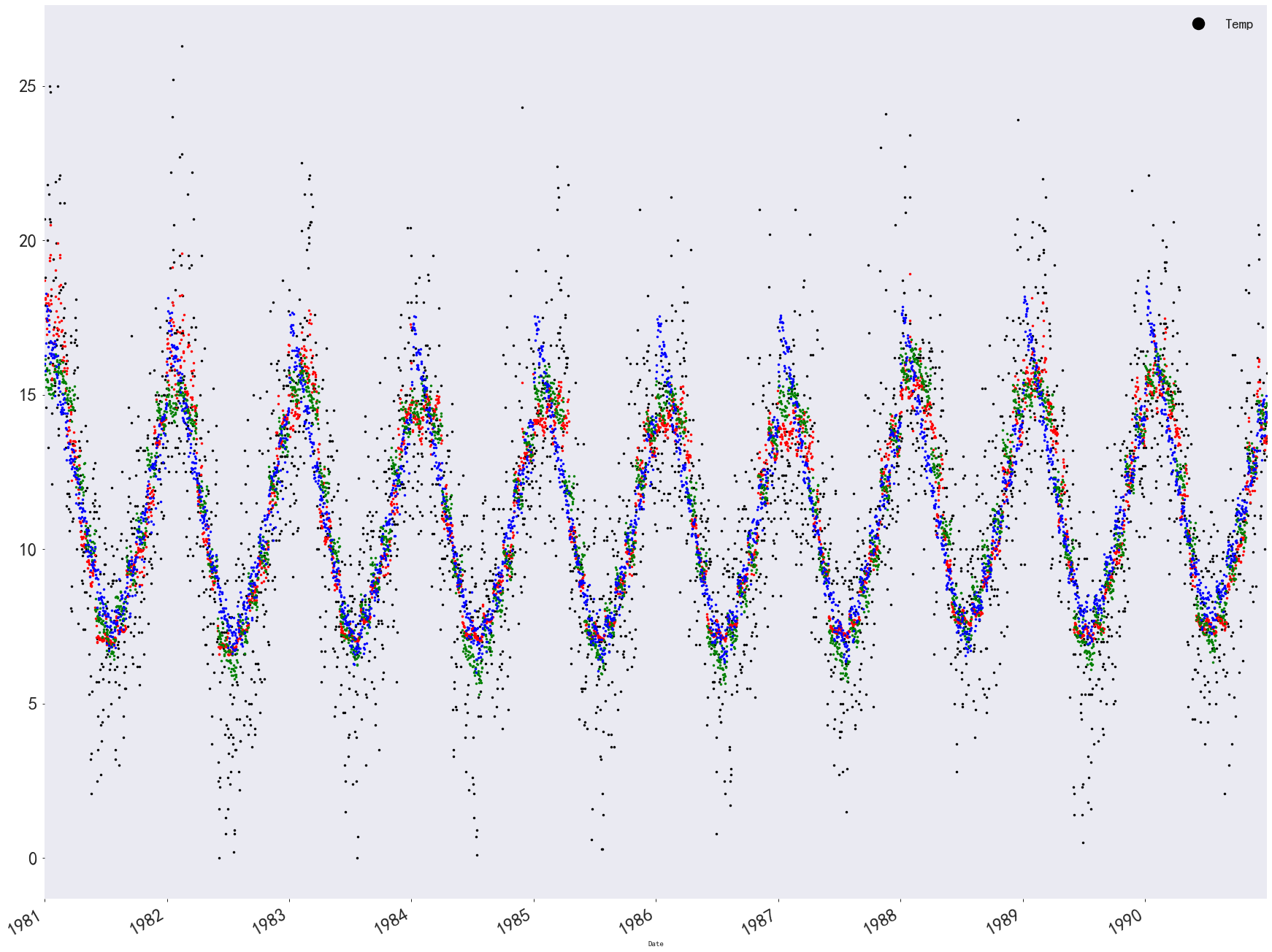7. 将回归拟合的结果文件保存，并比较不同模型的表现
df.to_csv('final_regression.csv', index=True)

result_analyse = result.describe().copy()
# 给result_analyse新构造一行MSE
result_analyse.loc['MSE', :] = 0

# 构造计算均方误差的函数
def MSE(yhat, y):
error = np.array(yhat-y)
error_power = np.power(error, 2)
MSE_error = np.sum(error_power) / len(y)

return MSE_error

# 把result_analyse新构造的MSE行填模型的均方误差
for each in result_analyse.columns:
result_analyse.loc['MSE', each] = MSE(result[each], result['Temp'])
result_analyse

结果如下：最后是一个模型比较的可视化结果：
plt.figure(figsize=(20, 20))
plt.subplot(421)
plt.title('平均值')
result_analyse.loc['mean', :].plot(kind='bar', color='k')

plt.subplot(422)
plt.title('方差')
result_analyse.loc['std', :].plot(kind='bar', color='y')

plt.subplot(423)
plt.title('最小值')
result_analyse.loc['min', :].plot(kind='bar', color='m')

plt.subplot(424)
plt.title('下四分位数')
result_analyse.loc['25%', :].plot(kind='bar', color='c')

plt.subplot(425)
plt.title('中位数')
result_analyse.loc['50%', :].plot(kind='bar', color='r')

plt.subplot(426)
plt.title('上四分位数')
result_analyse.loc['75%', :].plot(kind='bar', color='g')

plt.subplot(427)
plt.title('最大值')
result_analyse.loc['max', :].plot(kind='bar', color='b')

plt.subplot(428)
plt.title('均方误差')
result_analyse.loc['MSE', :].plot(kind='bar', color='deepskyblue')

plt.show()

这里展示一部分结果：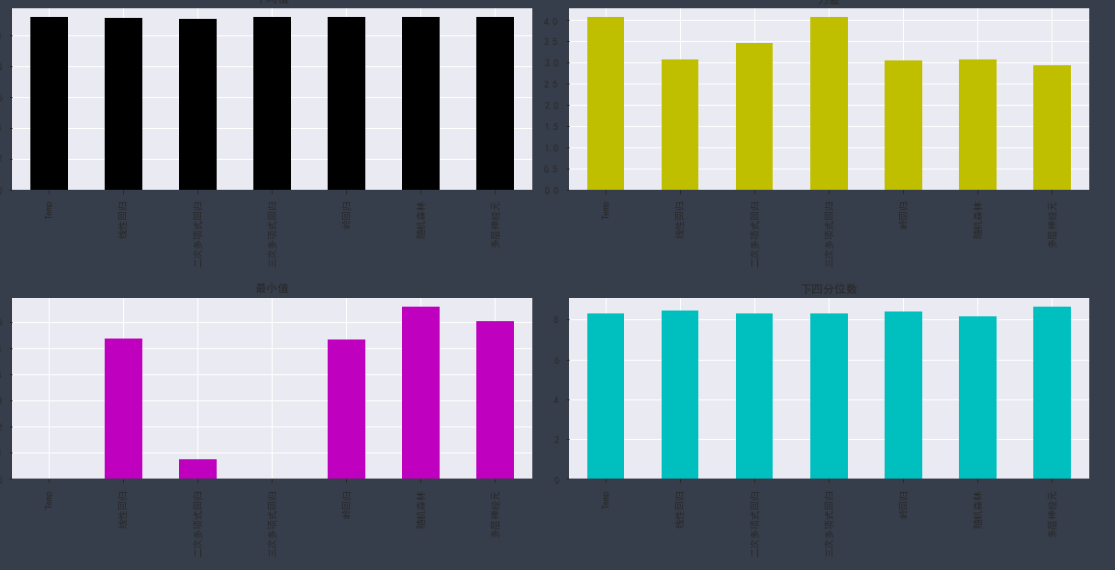8. 总结
这里只是想单纯的整理一个时间序列挖掘模板，方便以后查询使用，毕竟感觉写的还是挺全的，从数据导入，探索，各种格式化，到特征工程，建立各种模型，各种模型的评估等，我觉得在后面的任务中，会有借鉴之处，所以先整理下来。
如果想看详细的讲解视频： 时间序列数据挖掘与机器学习：墨尔本十年气温数据集 如果想获取详细的代码和数据集：https://download.csdn.net/download/wuzhongqiang/12245197
展开全文• ——平稳性的意义如何检验时间序列数据的平稳性？——单位根检验数据平稳怎么办？——协整检验单整、协整（cointegration）协整检验总结——时间序列数据的一般处理流程 什么要把时间序列变成平稳的？——平稳性...


时间序列的平稳性
为什么要把时间序列变成平稳的？——平稳性的意义如何检验时间序列数据的平稳性？——单位根检验数据不平稳怎么办？——协整检验单整、协整（cointegration）协整检验
总结——时间序列数据的一般处理流程

为什么要把时间序列变成平稳的？——平稳性的意义
凭以推测经济系统（或其相关变量）在未来可能出现的状况，亦即预测经济系统（或其相关变量）的走势，是我们建立经济计量模型的主要目的。而基于随机变量的历史和现状来推测其未来，则是我们实施经济计量和预测的基本思路。这就需要假设随机变量的历史和现状具有代表性或可延续性。换句话说，随机变量的基本特性必须能在包括未来阶段的一个长时期里维持不变。否则，基于历史和现状来预测未来的思路便是错误的。
样本时间序列展现了随机变量的历史和现状，因此所谓随机变量基本性态的维持不变也就是要求样本数据时间序列的本质特征仍能延续到未来。我们用样本时间序列的均值、方差、协（自）方差来刻画该样本时间序列的本质特征。于是，我们称这些统计量的取值在未来仍能保持不变的样本时间序列具有平稳性。可见，一个平稳的时间序列指的是：遥想未来所能获得的样本时间序列，我们能断定其均值、方差、协方差必定与眼下已获得的样本时间序列等同。也就是说，均值、方差、协方差必定为常数，不然就是不平稳的.
如何检验时间序列数据的平稳性？——单位根检验
注：在计量经济学中，检验数据的平稳性一般不使用原始数据，而是对原始数据取对数。取对数之后的数据可以理解为原始数据的变化率。例如，原始数据是中国年度GDP数据，log之后的数据表示中国GDP的年增长值。之所以取对数，是因为大多数原始数据都不平稳，例如GDP是一个上涨趋势的函数，肯定不平稳。所以，在检验平稳性之前，就取对数，再对原始数据的对数进行平稳性检验。
虽然定义经过d阶差分后是平稳的，但是软件只提供到2阶差分，若是原始数据没有经过差分就平稳，则说明那是零阶单整，记为I（0）的过程。
在stata中，单位根检验命令为：dfuller lnagdp
数据不平稳怎么办？——协整检验
单整、协整（cointegration）
单整
如果一个时间序列经过一次差分变成平稳的，则称原序列是1阶单整的，记为I（1）。 一般地，如果时间序列经过d次差分后变成平稳序列，而经过d-1次差分仍不平稳，则称原序列是d阶单整序列，记为I（d）。
协整
协整是指若两个或多个非平稳的变量序列，其某个线性组合后的序列呈平稳性。
数学上的解释是这样的：
特别当  和  都是一阶单整时，一般而言， 和  的线性组合  仍然是一阶单整的，但是对于某些非零向量  ，会使得 ，此时非零向量  称作协整向量，其中每一项   为 t 时刻的协整系数。通俗点说，如果两组序列都是非平稳的，但是经过一阶差分后是平稳的，且这两组序列经过某种线性组合也是平稳的，则它们之间就存在协整关系。
协整的直观解释： 协整是说两个或多个变量之间具有长期的稳定关系。但变量间协整的必要条件是它们之间是同阶单整，也就是说在进行协整检验之前必须进行单位根检验。
协整说的是变量之间存在长期的稳定关系，这只是从数量上得到的结论，但不能确定谁是因，谁是果。而因果关系检验解决的就是这个问题。
协整检验
协整检验的意义？——判断变量间是否存在长期的稳定关系 协整检验是用来分析变量之间的长期均衡关系,在协整分析两变量的过程中,如果自变量和因变量是协整的,我们就可以确信这两变量不会产生伪回归结果并且这两个变量存在长期稳定的关系。  协整检验的条件？——变量同阶单整 变量间协整的必要条件是它们之间是同阶单整，也就是说在进行协整检验之前必须进行单位根检验。
协整的要求或前提是同阶单整，但也有如下的宽限说法：如果变量个数多于两个，即解释变量个数多于一个，被解释变量的单整阶数不能高于任何一个解释变量的单整阶数。另当解释变量的单整阶数高于被解释变量的单整阶数时，则必须至少有两个解释变量的单整阶数高于被解释变量的单整阶数。如果只含有两个解释变量，则两个变量的单整阶数应该相同。
就是说，单整阶数不同的两个或以上的非平稳序列如果一起进行协整检验，必然有某些低阶单整的，即波动相对高阶序列的波动甚微弱（有可能波动幅度也不同）的序列，对协整结果的影响不大，因此包不包含的重要性不大。而相对处于最高阶序列，由于其波动较大，对回归残差的平稳性带来极大的影响，所以如果协整是包含有某些高阶单整序列的话（但如果所有变量都是阶数相同的高阶，此时也被称作同阶单整，这样的话另当别论），一定不能将其纳入协整检验。
注：同之前平稳性检验中相同，在协整检验中，一般不直接用原始数据，而是先对原始数据取对数。所以，虽然满足同阶单整的条件就可以进行协整检验，但在计量经济学的实证中，一般不做两次差分，最多一次差分。这是因为，两次差分没有实际的经济学意义（一次差分表示数据的变化值，在取对数的前提下，一次差分的结果表示变化率的变化值）。所以，在计量经济学实证中，通常是对以下两种情况进行协整检验：
被解释变量一阶单整，解释变量都一阶单整被解释变量一阶单整，解释变量一部分一阶单整，一部分平稳
总结——时间序列数据的一般处理流程
先做单位根检验，看变量序列是否平稳序列，若平稳，可构造回归模型等经典计量经济学模型；若非平稳，进行差分，当进行到第d次差分时序列平稳，则服从i阶单整。若所有检验序列均服从同阶单整，可构造VAR模型，做协整检验，判断模型内部变量间是否存在协整关系，即是否存在长期均衡关系。如果有，则可以构造VEC模型或者进行Granger因果检验，检验变量之间“谁引起谁变化”，即因果关系。
展开全文• ## 时间序列数据预测

千次阅读 2018-12-08 17:55:17
ts(data = NA, start = 1, end = numeric(), frequency = 1, ...单个数字或两个整数的向量，它们指定一个自然时间单位和进入时间单位的(基于1的)样本数量 end：最后一次观测的时间，用与开始相同的方...指数平均 arima
• ## python时间序列数据预测教程之 arima

千次阅读 热门讨论 2020-04-14 23:27:01
目前关于时间序列数据分析预测大致有三种主流方法： 1、ARIMA系列方法 2、facebook开源的Prophet模型 3、LSTM时间序列预测 本系列将用python进行实现并做出总结。 首先，本文项目中所用数据近一段时间内，间隔30...python
• ## 数据挖掘之时间序列分析

万次阅读 多人点赞 2018-08-12 23:45:16
按时间顺序排列的一组随机变量X1,X2,…,Xt表示一个随机事件的时间序列时间序列分析的目的是给定一个已被观测了的时间序列，预测该序列的未来值。 表1 常用的时间序列模型 模型名称 描述 平滑法 常...ARIMA
• 时间序列数据的时间间隔可以是分和秒（如高频金融数据），也可以是日、周、月、季度、以及甚至更大的时间单位。数据分析解决方案提供商 New Relic 在其博客上介绍了为时间序列数据优化 K-均值聚类速度的方法。机器...
• 时间序列数据是一个物体或多个物体随着时间不断产生的数值序列。比如：从智能电表中获得月电度使用量；每日股票的价格和交易量；ECG(心电图)；地震仪，网络性能数据等等。时间序列数据一定基于时间顺序，这个顺序是...大数据 人工智能 streams
• 大多数现代开源AutoML框架并没有广泛地涵盖时间序列预测任务。本文中我们将深入地研究AutoML框架之一FEDOT，它可以自动化时间序列预测的机器学习管道设计。因此，我们将通过时间序列预测的现实世界任务详细解释FEDOT...机器学习 automl
•  什么是时间序列（Time Series，以下简称时序）数据？从定义上来说，就是一串按时间维度索引的数据。用描述性的语言来解释什么是时序数据，简单的说，就是这类数据描述了某个被测量的主体在一个时间范围内的每个...
• 文章目录时间序列一.日期和时间数据类型及工具1.1字符串与datetime互相转换二.时间序列基础 时间序列 时间序列（time series）数据是一种重要的结构化数据形式，应用于多...时间序列数据的意义取决于具体的应用场景，主python
• 序列数据（具有时间依赖性的数据）在业务中非常常见，从信用卡交易到医疗保健记录再到股票市场价格。 但是，隐私法规限制并极大地减慢了对研发至关重要的有用数据的访问。 这就产生了对具有高度代表性但又完全私有的...机器学习 深度学习 生成对抗网络 人工智能
• 本文介绍了使用Python yield 实现“滑动窗口”处理序列数据的方法。深度学习 python yield
• 0 导读 阅读本文需要有掌握基本的ARIMA知识，倘若ARIMA相关内容已经遗忘，此处提供以下博文帮你回忆一下： 时间序列预测之--ARIMA模型 ...和许多时间序列分析一样，本文同样使用航空乘客数据（AirPassen...
• 用R分析时间序列(time series)数据 时间序列（time series）是一系列有序的数据。通常是等时间间隔的采样数据。如果不是等间隔，则一般会标注每个数据点的时间刻度。  下面time series 普遍使用的数据 ...R语言
• Python中的Pandas库为时间序列数据提供了出色的内置支持。 加载后，Pandas还提供了探索和更好地理解数据集的工具。 在本文中，您将了解如何加载和浏览时间序列数据集。 完成本教程后，您将了解： 如何使用Pandas...
• ## 时间序列

千次阅读 2018-09-02 00:33:43
所谓时间序列就是按照时间的顺序记录的一列有序数据。对时间序列进行观察、研究、找寻他发展变化的规律，预测他将来的走势就是时间序列分析，时间序列分析方法只适用于近期与短期的预测。 相关特征统计量： 均值...
• 今天要分析的一个kernel是一个关于elo的loyalty关于时间序列的关系的研究的kernel。 关于竞赛介绍及基础知识见： 我的上一篇内核分析：https://blog.csdn.net/ssswill/article/details/85217702、 这篇kernel来自...kaggle
• 转载：https://yq.aliyun.com/articles/104243摘要： 什么是时间序列数据   什么是时间序列（Time Series，以下简称时序）数据？从定义上来说，就是一...什么是时间序列数据  什么是时间序列（Time Series，...
• 时间序列的平稳性和单位根检验 内容包括时间序列的平稳性和单位根检验的讲述与具体的分析步骤。ppt格式的。
• 通过之前有关LSTM的8遍基础教程和10篇处理时间序列预测任务的教程介绍，使用简单的序列数据示例，已经把LSTM的原理，数据处理流程，模型架构，Keras实现都讲清楚了。从这篇文章开始，将介绍有关时间序列预测和时间...深度学习 tensorflow
• 研究时间序列主要目的：进行预测，根据已有的时间序列数据预测未来的变化。 时间序列预测关键：确定已有的时间序列的变化模式，并假定这种模式会延续到未来。 时间序列预测法的基本特点 假设事物发展趋势会...
• 时间序列数据挖掘（二）机器学习+统计学+kdd1718论文机器学习下的时间序列RNNRNN使用领域LSTM统计学下的时间序列ARIMAARIMA的含义模型前提：平稳ARIMA的数学形式ARIMA模型建立步骤一些细节 机器学习+统计学+kdd1718...
• 沃尔玛产品部门销售的时间序列分析和预测 项目介绍 在该项目中，我们小组根据来自的Walmart五年单位销售数据，使用四种模型对一种Walmart产品（FOODS_3_352）进行了28天单位销售预测。 首先，我们进行了基本的数据...HTML
• ## 时间序列预测算法总结

万次阅读 多人点赞 2018-10-18 10:30:48
time series data mining 主要包括decompose（分析数据的各个成分，例如趋势，周期性），prediction（预测未来的值），classification（对有序数据序列的feature提取与分类），clustering（相似数列聚类）等。...算法
• 7.14 处理时间序列 原文：Working with Time Series 译者：飞龙 协议：CC BY-NC-SA 4.0 本节是《Python 数据科学手册》（Python Data Science Handbook）的摘录。 Pandas 是在金融建模的背景下开发的，正如你所料...R语言...